# Matlab/Octave tools for geophysical studies

François Beauducel

Matlab/Octave scripts for geophysical studies and others.
All are open-source codes, working with Matlab core (no Toolbox needed) or free GNU Octave, shared through Mathworks Matlab Central File Exchange (best rank = 50)
Institut de Physique du Globe de Paris, 1993-2014

## Deformation analytical models summary

ModelDescriptionParametersOutputsReferences
ΔVsurface (**)
ΔVsource
SpaceSourceMedium
Mogi Point source in elastic half-space
Approximation for sphere of radius a << f
r f, ΔV
f, ΔP, a
ν
E, ν or μ
displacements, tilt, strains at surface [Anderson, 1936]
[Mogi, 1958]
2.(1 – ν)
= 1.5
Sun Penny-shaped crack in elastic half-space
Approximation for h/a >> 1
r h, a, ΔV
h, a, ΔP

E, ν
displacements at surface [Sun, 1969]
1
Okada Rectangular dislocation in elastic half-space x,y d, δ, θ, W, L
R, USLIP, UOPEN
ν displacements, tilt, strains at surface [Okada, 1985]
 1 – 1 – 2ν . sin²δ 2
= 1 (sill)
= 0.75 (dyke)
Okubo Rectangular dislocation in elastic half-space x,y d, δ, θ, W, L
R, USLIP, UOPEN
ν, ρ, ρ' gravity and elevation change at surface [Okubo, 1992]
(**) after [Delaney & McTigue, 1994]. Numerical values stand for isotropic medium (ν = 0.25)
r Radial distance from source X-coordinate from source Y-coordinate from source Focal depth of source Radius of source Depth of top-edge fault Width of fault Length of fault Dip-angle of fault Strike-angle of fault (azimut) Volume variation of source Pressure variation of source Rake-angle of dislocation Slip-dislocation (in Rake-angle direction) Tensile-dislocation (opening) Rigidity of medium (Lamé's second parameter) Lamé's first parameter Elasticity of medium (Young's modulus) Poisson's ratio of medium Density of medium Density of cavity-filling matter

Some conversion formulas between elastic parameters λ, μ, E and ν:

 λ λ+2μ
=
 ν 1–ν
ν =
 λ 2(λ+μ)
=
 E 2μ
– 1
E =
 μ(3λ+2μ) λ+μ
=
 λ(1+ν)(1–2ν) ν
= 2μ(1+ν)
λ =
 μ(E–2μ) 3μ–E
=
 Eν (1+ν)(1–2ν)
=
 2μν 1–2ν
μ =
 λ(1–2ν) 2ν
=
 E 2(1+ν)

## MOGI: point source in elastic half-space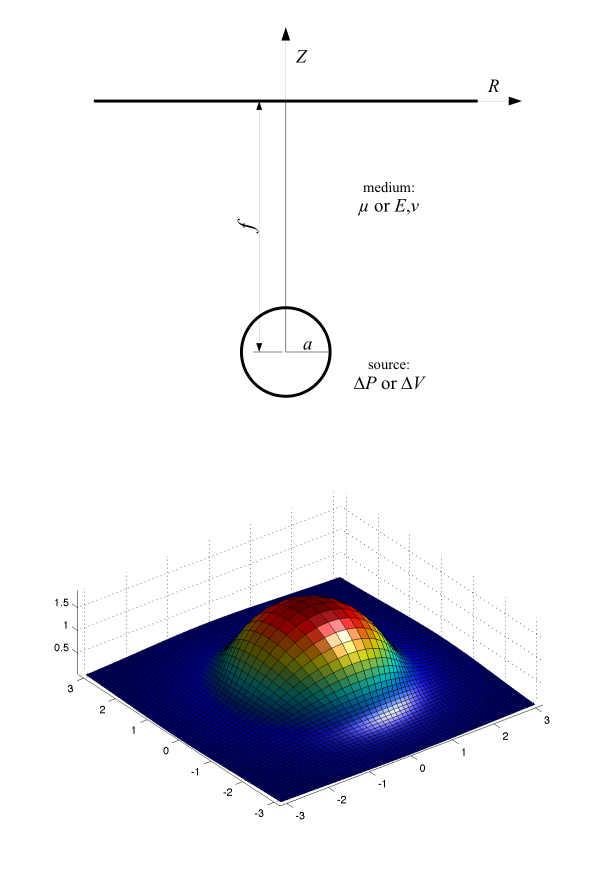The Mogi  model calculates analytical solution for surface deformation due to a point source in an elastic half-space. This model is widely used to simulate ground deformation produced by local perturbation like volcanic magma chamber. It computes displacements, tilt and strain in a polar space, due to a volume variation at depth [Anderson, 1936] or an isotropic pressure variation in a spherical source [Mogi, 1958]. The proposed Matlab script is a literal transcription of Mogi's simple equations, extended to non-isotropic medium (Poisson's ratio different from 0.25). All parameters can be vectorized. See help for syntax, and script comments for details. ```MOGI Mogi's model (point source in elastic half-space). [Ur,Uz,τ,σr,σt] = MOGI(R,F,ΔV,ν) or MOGI(R,F,A,ΔP,E,ν) computes radial and vertical displacements Ur and Uz, ground tilt τ, radial and tangential strain σr and σt on surface, at a radial distance R from the top of the source due to a hydrostatic pressure inside a sphere of radius A at depth F, in a homogeneous, semi-infinite elastic body and approximation for A << (center of dilatation). Formula by Anderson  and Mogi . MOGI(R,F,ΔV) and MOGI(R,F,A,μ,ΔP) are also allowed for compatibility (Mogi's original equation considers an isotropic material with Lamé constants equal, i.e., λ = μ, ν = 0.25). Input variables are: F: depth of the center of the sphere from the surface, ΔV: volumetric change of the sphere, A: radius of the sphere, ΔP: hydrostatic pressure change in the sphere, E: elasticity (Young's modulus), ν: Poisson's ratio, μ: rigidity (Lamé constant in case of isotropic material). Notes: - Equations are all vectorized, so variables R,F,V,A,μ and P can be vectors or N-D matrix of the same size and any of them can be scalars; then outputs will be also vector or matrix. - Convention: Uz > 0 = UP, F is depth so in -Z direction. - Units should be constistent, e.g.: R, F, A, Ur and Uz in m imply ΔV in m³; E, μ and P in Pa; τ in rad, σr, σt and ν dimensionless. Example for a 3-D plot of exagerated deformed surface due to a 1-bar overpressure in a 10-cm radius sphere at 1-m depth in rock: [x,y] = meshgrid(-3:.1:3); [th,rho] = cart2pol(x,y); [ur,uz] = mogi(rho,1,0.1,1e5,10e9,0.25); [ux,uy] = pol2cart(th,ur); ps = 1e8; surf(x+ux*ps,y+uy*ps,uz*ps), axis equal, light Author: François Beauducel Institut de Physique du Globe de Paris Created: 1997 Updated: 2012-04-05 References: Anderson, E.M., Dynamics of the formation of cone-sheets, ring-dikes, and cauldron-subsidences, Proc. R. Soc. Edinburgh, 56, 128-157, 1936. Mogi, K., Relations between the eruptions of various volcanoes and the deformations of the ground surfaces around them, Bull. Earthquake Res. Inst. Univ. Tokyo, 36, 99-134, 1958. Acknowledgments: Raphaël Grandin, Benoît Taisne ``` Download mogi.m at Matlab Central file exchange (Copyright 1997-2012 F. Beauducel / BSD License).

## SUN: Deformation from penny-shaped crack in elastic half-space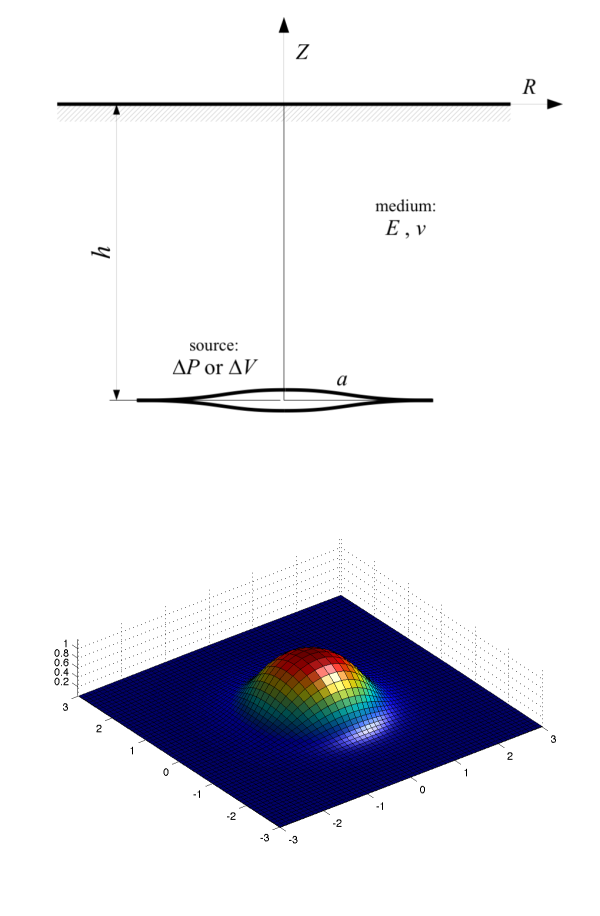The Sun  model calculates analytical solution for surface deformation due to hydrostatic pressure inside a horizontal circular fracture (“penny-shaped”) in an elastic half-space. The proposed Matlab script is a literal transcription of the Sun's paper equations. The equations are also vectorized for all input parameters. See help for syntax, and script comments for details. ```SUN69 Deformation from penny-shaped crack in elastic half-space. [Ur,Uz] = SUN69(R,H,A,ΔV) or SUN69(R,H,A,ΔP,E,ν) computes radial and vertical displacements Ur and Uz on the free surface, due to a horizontal circular fracture formed in a semi-infinite elastic medium, with following variables: R: radial distance of observation, H: depth of the center of the source from the surface, A: radius of the source with the hydrostatic pressure, ΔV: volume of injected material, ΔP: change of the hydrostatic pressure in the crack. E: Young's modulus, ν: Poisson's ratio (default is 0.25 for isotropic medium). [Ur,Uz,B] = SUN69(...) returns also the maximum separation of fracture (vertical displacement) B. Equations from Sun , with approximation H/A >> 1. If H/A > 2, error is about 2 to 3%; if H/A > 5, solution is almost perfect. Notes: - Equations are all vectorized, so variables R,H,A,ΔV or ΔP can be scalar or any of them vector or matrix, then outputs will have the same size. - Convention: Uz > 0 = UP, H is depth so in -Z direction. - Units should be constistent, e.g.: R, H, A, Ur and Uz in m imply V in m³; optional E and ΔP in Pa, ν dimensionless. Example for a 3D plot of exagerated deformed surface: [x,y] = meshgrid(-3:.1:3); [th,rho] = cart2pol(x,y); [ur,uz] = sun69(rho,1,.5,1e6,10e9,0.25); [ux,uy] = pol2cart(th,ur); ps = 5e4; surf(x+ux*ps,y+uy*ps,uz*ps), axis equal, light Author: François Beauducel Institut de Physique du Globe de Paris, 2009. References: Sun, R. J. (1969). Theoretical size of hydraulically induced horizontal fractures and corresponding surface uplift in an idealized medium, J. Geophys. Res., 74, 5995-6011. ``` Download sun69.m at Matlab Central file exchange (Copyright 2010 F. Beauducel / BSD License).

## OKADA: Surface deformation due to a finite rectangular source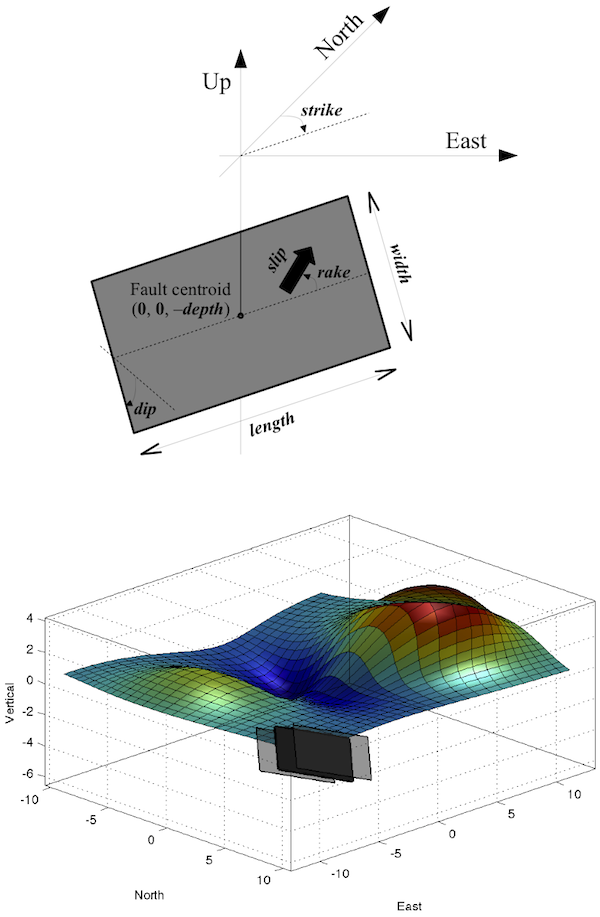The Okada  model calculates analytical solution for surface deformation due to shear and tensile faults in an elastic half-space. This model is widely used to simulate ground deformation produced by local perturbation like tectonic faults (earthquakes) or volcanic dykes (magmatic intrusion). Given rectangular fault geometry (length, width, depth, strike, dip) and 3-component dislocation amplitude (rake, slip and open), it computes the displacements, tilts and strains at the free-surface. The proposed Matlab script is a literal transcription of the Okada's equations, except that it is transposed in a geographical referential (East, North, Up), where the fault is defined by a strike angle relative to the North, and dislocation parameters are given by: rake, slip and opening (instead of U1, U2, U3), following Aki & Richards  definition. All coordinates and depth are relative to fault centroid. Lamé's constants λ and μ are replaced by Poisson's ratio ν (with a default value of 0.25 for isotropic medium), since the equations are independent of other elastic parameters. The equations are also vectorized for (x,y) coordinates and all input parameters except dip angle. To check the consistency of numerical calculations, run the script okada85_checklist.m, a transcription of table 2 cases 2, 3, and 4 checklist from [Okada, 1985] paper. See help for further details, syntax, example, and script comments for technical details. ```OKADA85 Surface deformation due to a finite rectangular source. [uE,uN,uZ,uZE,uZN,uNN,uNE,uEN,uEE] = OKADA85(... E,N,DEPTH,STRIKE,DIP,LENGTH,WIDTH,RAKE,SLIP,OPEN) computes displacements, tilts and strains at the surface of an elastic half-space, due to a dislocation defined by RAKE, SLIP, and OPEN on a rectangular fault defined by orientation STRIKE and DIP, and size LENGTH and WIDTH. The fault centroid is located (0,0,-DEPTH). E,N : coordinates of observation points in a geographic referential (East,North,Up) relative to fault centroid (units are described below) DEPTH : depth of the fault centroid (DEPTH > 0) STRIKE : fault trace direction (0 to 360° relative to North), defined so that the fault dips to the right side of the trace DIP : angle between the fault and a horizontal plane (0 to 90°) LENGTH : fault length in the STRIKE direction (LENGTH > 0) WIDTH : fault width in the DIP direction (WIDTH > 0) RAKE : direction the hanging wall moves during rupture, measured relative to the fault STRIKE (-180 to 180°) SLIP : dislocation in RAKE direction (length unit) OPEN : dislocation in tensile component (same unit as SLIP) returns the following variables (same matrix size as E and N): uN,uE,uZ : displacements (unit of SLIP and OPEN) uZE,uZN : tilts (in rad * FACTOR) uNN,uNE,uEN,uEE : horizontal strains POSITIVE = COMPRESSION (unit of FACTOR) Length unit consistency: E, N, DEPTH, LENGTH, and WIDTH must have the same unit (e.g. km) which can be different from that of SLIP and OPEN (e.g. m) but with a possible FACTOR on tilt and strain results (in this case, an amplification of km/m = 1000). To have FACTOR = 1 (tilt in radians and correct strain unit), use the same length unit for all aforesaid variables. [...] = OKADA85(...,NU) specifies Poisson's ratio NU (default is 0.25 for an isotropic medium). Formulas and notations from Okada  solution excepted for strain convention (here positive strain means compression), and for the fault parameters after Aki & Richards , e.g.: DIP=90, RAKE=0 : left lateral (senestral) strike slip DIP=90, RAKE=180 : right lateral (dextral) strike slip DIP=70, RAKE=90 : reverse fault DIP=70, RAKE=-90 : normal fault It is also possible to produce partial outputs, with following syntax: [uE,uN,uZ] = OKADA85(...) for displacements only; [uE,uN,uZ,uZE,uZN] = OKADA85(...) for displacements and tilts; [uE,uN,uZ,uNN,uNE,uEN,uEE] = OKADA85(...) for displacements and strains; [uZE,uZN] = OKADA85(...) for tilts only; [uZE,uZN,uNN,uNE,uEN,uEE] = OKADA85(...) for tilts and strains; [uNN,uNE,uEN,uEE] = OKADA85(...) for strains only. Note that vertical strain components can be obtained with following equations: uNZ = -uZN; uEZ = -uZE; uZZ = -(uEE + uNN)*NU/(1-NU); [...] = OKADA85(...,'plot') or OKADA85(...) without output argument produces a 3-D figure with fault geometry and dislocation at scale (if all of the fault parameters are scalar). Equations are all vectorized excepted for argument DIP which must be a scalar (beacause of a singularity in Okada's equations); all other arguments can be scalar or matrix of the same size. Example: [E,N] = meshgrid(linspace(-10,10,50)); [uE,uN,uZ] = okada85(E,N,2,30,70,5,3,-45,1,1,'plot'); figure, surf(E,N,uN) considers a 5x3 fault at depth 2, N30° strike, 70° dip, and unit dislocation in all directions (reverse, senestral and open). Displacements are computed on a regular grid from -10 to 10, and North displacements are plotted as a surface. Author: François Beauducel Institut de Physique du Globe de Paris Created: 1997 Updated: 2014-05-24 References: Aki K., and P. G. Richards, Quantitative seismology, Freemann & Co, New York, 1980. Okada Y., Surface deformation due to shear and tensile faults in a half-space, Bull. Seismol. Soc. Am., 75:4, 1135-1154, 1985. Acknowledgments: Dmitry Nicolsky, Qian Yao, Halldor Geirsson. ``` ATTENTION: new version on May 24, 2014 with bug corrections. Update your file! Download okada85.m at Matlab Central file exchange (Copyright 1997-2014 F. Beauducel / BSD License). See also the PLUME Project page (in French).

## OKUBO: Gravity change due to shear and tensile faults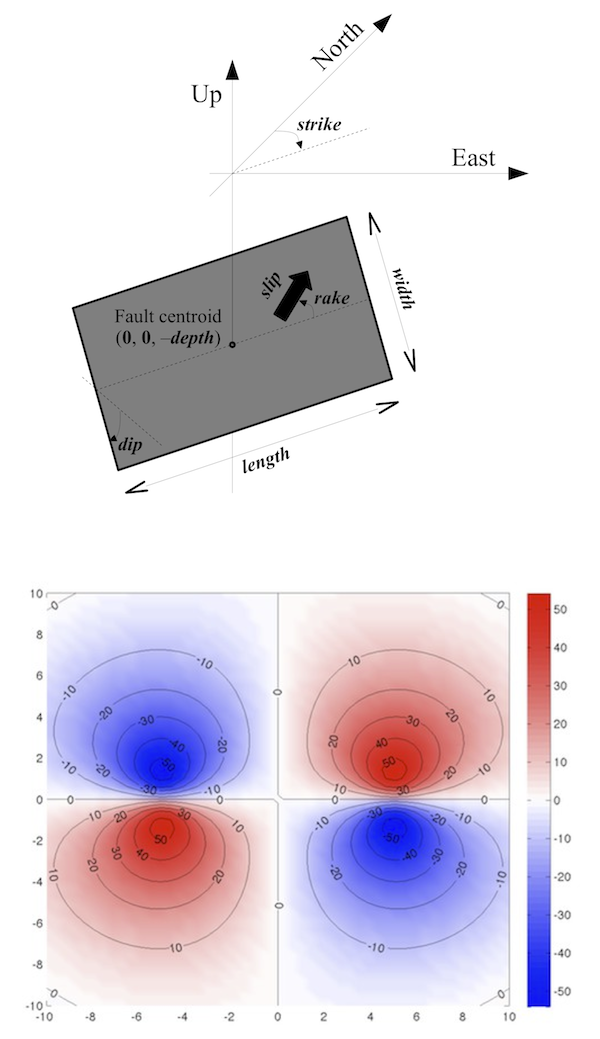The Okubo  model calculates analytical solution for gravity changes due to shear and tensile faults in an elastic half-space (as Okada  does for deformations). This model can be used to simulate gravity anomalies produced by local perturbation like tectonic faults (earthquakes) or volcanic dykes (magmatic intrusion). Given rectangular fault geometry (length, width, depth, strike, dip) and 3-component dislocation amplitude (rake, slip and open), it computes the total gravity change (potential, cavity filling and free-air) at the free-surface. The proposed Matlab script is a literal transcription of the Okubo's equations, except for the fault geometry that it is transposed in a geographical referential (East, North, Up), where the fault is defined by a strike angle relative to the North, and dislocation parameters are given by: rake, slip and opening (instead of U1, U2, U3), following Aki & Richards  definition. All coordinates and depth are relative to fault centroid. The equations are also vectorized for (x,y) coordinates and all parameters except dip angle. Type "doc okubo92" for help, syntax and example, and see script comments for details. ```OKUBO92 Surface gravity change due to a finite rectangular source. [dG,dH] = OKUBO92(E,N,DEPTH,STRIKE,DIP,LENGTH,WIDTH,RAKE,SLIP,OPEN,RHO,RHOP) computes total gravity change at the free surface of an elastic half-space, due to a dislocation defined by RAKE, SLIP, and OPEN on a rectangular fault defined by orientation STRIKE and DIP, and size LENGTH and WIDTH. The fault centroid is located (0,0,-DEPTH). E,N : coordinates of observation points in a geographic referential (East,North,Up) relative to fault centroid (units are described below) DEPTH : depth of the fault centroid (DEPTH > 0) STRIKE : fault trace direction (0 to 360° relative to North), defined so that the fault dips to the right side of the trace DIP : angle between the fault and a horizontal plane (0 to 90°) LENGTH : fault length in the STRIKE direction (LENGTH > 0) WIDTH : fault width in the DIP direction (WIDTH > 0) RAKE : direction the hanging wall moves during rupture, measured relative to the fault STRIKE (-180 to 180°). SLIP : dislocation in RAKE direction (in m) OPEN : dislocation in tensile component (in m) RHO : density of the medium (in kg/m^3) RHOP : optional density of the cavity-filling matter (in kg/m^3); takes RHO value if not specified. returns the following variables (same matrix size as E and N): dG : total gravity change (in m/s^2) dH : elevation changes as given by Okada  model (in m) Length unit consistency: E, N, DEPTH, LENGTH, and WIDTH must have the same unit (e.g. km) which can be different from that of SLIP and OPEN (e.g. m to to produce dG in m/s^2 and dH in m). [...] = OKUBO92(...,RHOP,BETA,NU) specifies gravity vertical gradient BETA (default is 0.309e-5 s^-2) and Poisson's ratio NU (default is 0.25 for an isotropic medium). Formulas and notations from Okubo  solution excepted for the fault geometry parameters after Aki & Richards , e.g.: DIP=90, RAKE=0 : left lateral (senestral) strike slip DIP=90, RAKE=180 : right lateral (dextral) strike slip DIP=70, RAKE=90 : reverse fault DIP=70, RAKE=-90 : normal fault Equations are all vectorized excepted for argument DIP which must be a scalar; all other arguments can be scalar or matrix of the same size. Example: [E,N] = meshgrid(linspace(-10,10,50)); dG = okubo92(E,N,6,90,90,10,10,0,5,0,2670); figure, pcolor(E,N,1e8*dG), shading interp hold on, [c,h]=contour(E,N,1e8*dG,-50:10:50,'k'); clabel(c,h), hold off colorbar, polarmap reproduces the figure 4a in Okubo  paper, considering a 10x10km fault at 6km depth, oriented N90°-strike, 90°-dip, and 5m senestral dislocation. Gravity changes are computed on a regular grid from -10 to 10km, and are plotted as a surface in 1e-8 m/s^2 unit. POLARMAP is an author's colormap. See also OKADA85 author's function to compute complete displacements, tilt and strain at free surface. Author: Francois Beauducel Institut de Physique du Globe de Paris Created: 2012-06-11 Updated: 2012-06-12 References: Aki K., and P. G. Richards, Quantitative seismology, Freemann & Co, New York, 1980. Okubo S., Gravity and Potential Changes due to Shear and Tensile Faults in a Half-Space, J. Geophys. Res., 97:B5, 7137-7144, 1992. ``` Download okubo92.m at Matlab Central file exchange (Copyright 2012 F. Beauducel / BSD License).

## RDMSEED and MKMSEED: Read and write miniSEED files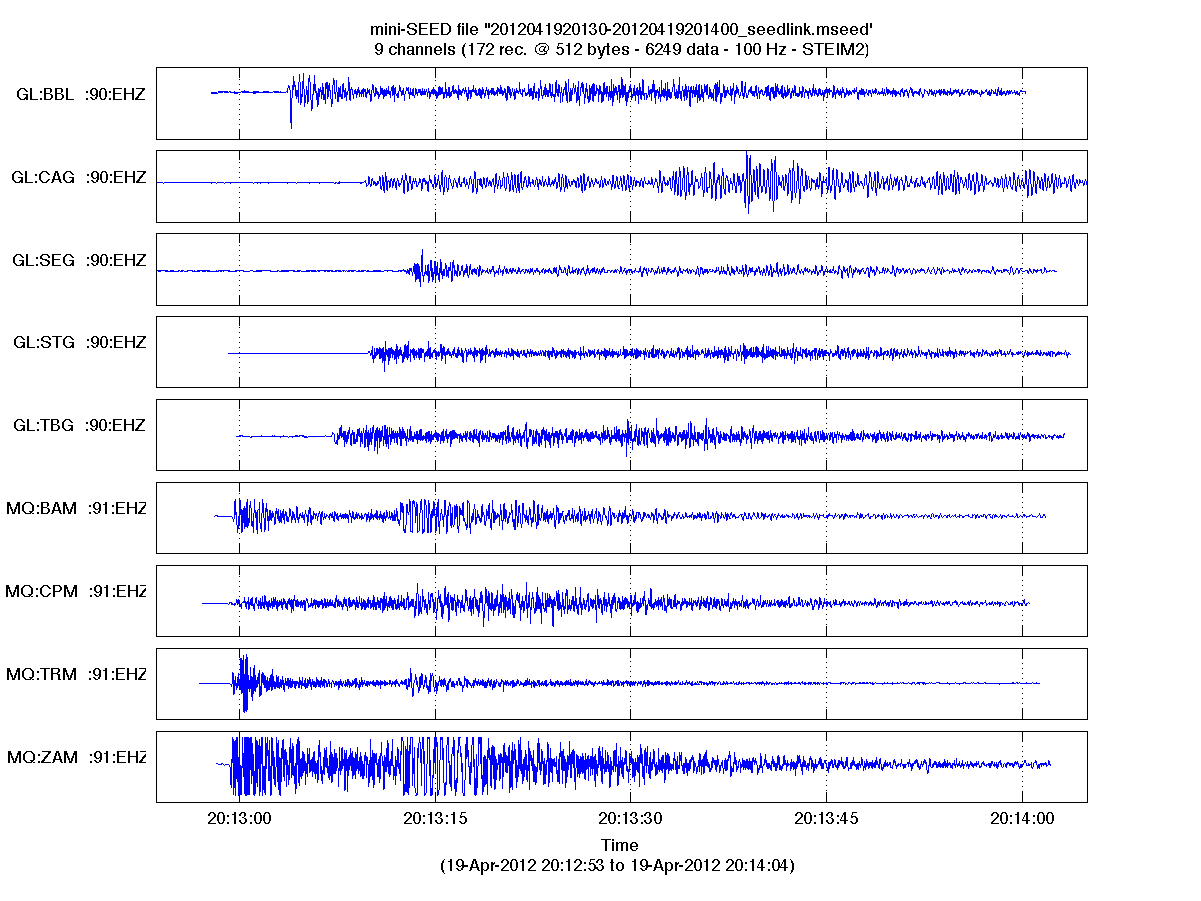The Standard for the Exchange of Earthquake Data (SEED) is an international standard format for the exchange of digital seismological data. SEED was designed for use by the earthquake research community, primarily for the exchange between institutions of unprocessed earth motion data. It is a format for digital data measured at one point in space and at equal intervals of time. The SEED format consists of Volume Control Headers, Abbreviation Control Headers, Station Control Headers, Time Span Control Headers and finally Data Records. In complement to “Dataless” SEED volumes, exists the “Data-only” volume called Mini-SEED (see http://www.iris.edu for further information). The purpose of these functions is to read and write miniSEED data files directly from Matlab, avoiding intermediate format conversion (like SAC or other formats for which many functions exist), having a full control on headers and formats. Note that IRIS proposes a complete C-library for miniSEED (libmseed) and there is a free Matlab toolbox using this library to read and write miniSEED files (see Stefan Mertl site), but it needs some compilation to be used (unsignificant for geeks, quite easy on UNIX/Linux, but no doubt more problematic for Windows users...). The present functions rdmseed.m and mkmseed.m are 100% Matlab code standalone scripts, slower, but fully portable and platform independent. rdmseed: reading miniSEED file Each data record is imported into a structure array, allowing to adress data blocks and header fields individually (useful for multi-channel files), just as concatenating all data with a simple cat(1,X.d) function. Time stamps are also converted into Matlab datenum format. The function reads miniSEED "data-only" using the two mostly used compression formats Steim-1 and Steim-2. General FDSN formats have also been implemented (ASCII, 16/24/32-bit integers, IEEE floats and doubles), and GEOSCOPE multiplexed old formats (24-bit, 16/3 or 16/4-bit gain ranged). All these formats should work but some of them have not been tested using real data. I also partly coded Steim-3 format but without a clear description and any file example... Since I never met any data file using this format, I don't know if it's really useful. The function detects also automatically big/little-endian coded files. Known Blockettes are 1000, 1001, 100, 500 and 2000. If there is no Blockette 1000 (which is mandatory in SEED format...), default 4096-byte record length, big-endian and Steim-1 compression are used. These values can be set using additional arguments. Using extra output argument, some analysis can be done on the data stream (detection of gaps and overlaps), and channel components are detected. Without any output arguments, or with an additionnal 'plot' input argument, the function plots the imported data in a new figure (works also in case of multi-channel file). Steim-1/2 compression decoding strategy has been deeply optimized for Matlab. The proposed method, as vectorized as possible, is about 30 times faster than a 'C-like' loops coding... which is still 10 times slower than the same C-compiled program, but, well, this is the Matlab's other side of the coin! mkmseed: writing miniSEED file The function allows to export a data vector D to miniSEED file, giving origin date and time T0 and sampling rate FS (for monotonic data) or a time vector T. Header information is specified using the filename string with conventional naming "Network.Station.Location.Channel". Output file names will have appended ".Year.Day" and multiple file may be produced if data exceed a day. Data encoding format can be specified (16/32-bit integers, IEEE float/double, Steim-1/2; Geoscope 16/3-4). If not, it will depend on the class of variable D. Note that D will be converted to relevant class depending on encoding format. Be aware that Steim-2 compression uses 30-bit signed integer to code difference between 2 consecutive samples; equivalent to a 29-bit coding. Steim-1 uses 32-bit signed integers to code differences, so a 31-bit equivalent. This means that full range of signed 32-bit integer data cannot be correctly encoded with these formats in some exceptional cases (may lead to data loss). Finally, Steim-1/2 encoding needs time processing to achieve a maximum compression ratio of about 6 in file size, while other formats are very fast but result in large files. Binary file will be big-endian coded, with blockettes 1000, and default record length is 4096 bytes (this may be changed using input argument). Type "doc rdmseed" or "doc mkmseed" for detailed usage. ```RDMSEED Read miniSEED format file. X = RDMSEED(F) reads file F and returns a M-by-1 structure X containing M blocks ("data records") of a miniSEED file with headers, blockettes, and data in dedicated fields, in particular, for each data block X(i): t: time vector (DATENUM format) d: data vector (double) BLOCKETTES: existing blockettes (substructures) Known blockettes are 100, 500, 1000, 1001 and 2000. Others will be ignored with a warning message. X = RDMSEED(F,ENCODINGFORMAT,WORDORDER,RECORDLENGTH), when file F does not include the Blockette 1000 (like Seismic Handler outputs), specifies: - ENCODINGFORMAT: FDSN code (see below); default is 10 = Steim-1; - WORDORDER: 1 = big-endian (default), 0 = little-endian; - RECORDLENGTH: must be a power of 2, at least 256 (default is 4096). If the file contains Blockette 1000 (which is mandatory in the SEED convention...), these 3 arguments are ignored. X = RDMSEED without input argument opens user interface to select the file from disk. [X,I] = RDMSEED(...) returns a N-by-1 structure I with N the detected number of different channels, and the following fields: ChannelFullName: channel name, XBlockIndex: channel's vector index into X, ClockDrift: vector of time interval errors, in seconds, between each data block (relative to sampling period). This can be compared to "Max Clock Drift" value of a Blockette 52. = 0 in perfect case < 0 tends to overlapping > 0 tends to gapping OverlapBlockIndex: index of blocks (into X) having a significant overlap with previous block (less than 0.5 sampling period). OverlapTime: time vector of overlapped blocks (DATENUM format). GapBlockIndex: index of blocks (into X) having a significant gap with next block (more than 0.5 sampling period). GapTime: time vector of gapped blocks (DATENUM format). RDMSEED(...) without output arguments plots the imported signal by concatenating all the data records, in one single plot if single channel is detected, or subplots for multi-channels file. Gaps are shown with red stars, overlaps with green circles. [...] = RDMSEED(F,...,'be') forces big-endian reading (overwrites the automatic detection of endianness coding, which fails in some cases). [...] = RDMSEED(F,...,'plot') forces the plot with output arguments. [...] = RDMSEED(F,...,'v') uses verbose mode (displays additional information and warnings when necessary). Use 'vv' for extras, 'vvv' for debuging. Some instructions for usage of the returned structure: - to get concatenated time and data vectors from a single-channel file: X = rdmseed(f,'plot'); t = cat(1,X.t); d = cat(1,X.d); - to get the list of channels in a multi-channel file: [X,I] = rdmseed(f); cat(1,I.ChannelFullName) - to extract the station component i from a multi-channel file: [X,I] = rdmseed(f); k = I(i).XBlockIndex; plot(cat(1,X(k).t),cat(1,X(k).d)) datetick('x') title(I(i).ChannelFullName) Known encoding formats are the following FDSN codes: 0: ASCII 1: 16-bit integer 2: 24-bit integer (untested) 3: 32-bit integer 4: IEEE float32 5: IEEE float64 10: Steim-1 11: Steim-2 12: GEOSCOPE 24-bit (untested) 13: GEOSCOPE 16/3-bit gain ranged 14: GEOSCOPE 16/4-bit gain ranged 19: Steim-3 (alpha and untested) See also MKMSEED to export data in miniSEED format. Author: François Beauducel Institut de Physique du Globe de Paris Created: 2010-09-17 Updated: 2014-05-07 Acknowledgments: Ljupco Jordanovski, Jean-Marie Saurel, Mohamed Boubacar, Jonathan Berger, Shahid Ullah, Wayne Crawford, Constanza Pardo. References: IRIS (2010), SEED Reference Manual: SEED Format Version 2.4, May 2010, IFDSN/IRIS/USGS, http://www.iris.edu Trabant C. (2010), libmseed: the Mini-SEED library, IRIS DMC. Steim J.M. (1994), 'Steim' Compression, Quanterra Inc. ``` ```MKMSEED Write data in miniSEED file format. MKMSEED(FILENAME,D,T0,FS) writes miniSEED file FILENAME from stritl monotonic data vector D, time origin T0 (a scalar in Matlab datenum compatible format) and sampling rate FS (in Hz). Encoding format will depend on D variable class (see below). MKMSEED(FILENAME,D,T,FS) where T is a time vector of the same length as data vector D, will create data records of monotonic blocks of samples, splitting each time the sampling frequency FS is not equal to time difference between two successive samples (with a 50% tolerency). Network, Station, Channel and Location codes will be extracted from FILENAME which must respect the format "NN.SSSSS.LC.CCC" where: NN = Network Code (2 characters max, see FDSN list) SSSSS = Station identifier (5 char max) LC = Location Code (2 char max) CCC = Channel identifier (3 char max) Final filename will have appended string ".YYYY.DDD" corresponding to year and ordinal day of origin time (from T0 value). Multiple files may be created if time span of data exceeds day limit. MKMSEED(...,EF,RL) specifies encoding format EF and data record length RL (in bytes). RL must be a power of 2 greater or equal to 256. Data encoding format EF must be one of the following FDSN codes: 1 = 16-bit integer (default for class 2-bit, 8-bit, int16) 3 = 32-bit integer (default for class uint16, int32) 4 = IEEE float32 (default for class single) 5 = IEEE float64 (default for all other class) 10 = Steim-1 compression (D will be converted to int32) 11 = Steim-2 compression (D will be converted to int32) 13 = Geoscope 16-3 gain ranged (D will be converted to double) 14 = Geoscope 16-4 gain ranged (D will be converted to double) MKMSEED(FILENAME,D) uses default value for T0 = now (present date and time), and FS = 100 Hz. File(s) will be coded big-endian, flags set to zero, blockette 1000, default record length is 4096 bytes. Outputs have been tested with PQL II software from IRIS PASSCAL (v2010.268). See also RDMSEED function for miniSEED file reading. Acknowledgments: Florent Brenguier, Julien Vergoz, Constanza Pardo. References: IRIS (2010), SEED Reference Manual: SEED Format Version 2.4, May 2010, IFDSN/IRIS/USGS, http://www.iris.edu IRIS (2010), PQL II Quick Look trace viewing application, PASSCAL Instrument Center, http://www.passcal.nmt.edu/ Author: François Beauducel Institut de Physique du Globe de Paris Created: 2011-10-19 Updated: 2014-05-07 ``` ATTENTION: new version of rdmseed on April 21, 2012 with bug corrections (little-endian coding). Update your file! Download rdmseed.m and mkmseed.m at Matlab Central file exchange (Copyright 2014 F. Beauducel / BSD License).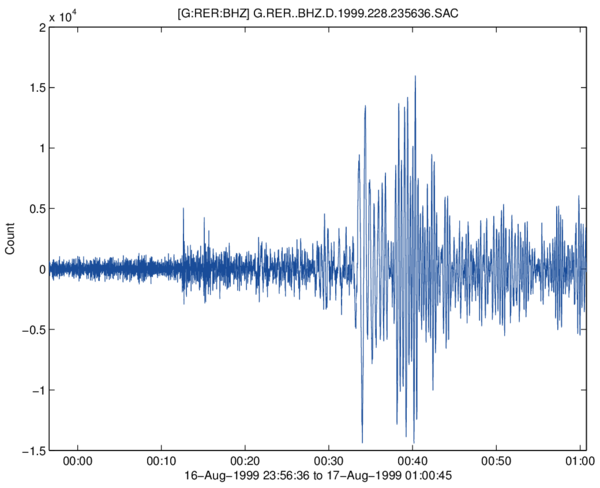RDSAC reads a seismic data file encoded in the IRIS/SAC binary format, and returns a time vector, a data vector and all header variables in a structure (field names correspond to exact IRIS variable names). Type "doc rdsac" for detailed usage. ```RDSAC Read SAC data file. X=RDSAC(FILE) reads the Seismic Analysis Code (SAC) FILE and returns a structure X containing the following fields: t: time vector (DATENUM format) d: data vector (double) HEADER: header sub-structure (as defined in the IRIS/SAC format). RDSAC without input argument will open a file browser window. X=RDSAC(...,'plot') or RDSAC(...) without output argument will plot the data in a new figure. Reference: http://www.iris.edu/files/sac-manual/ Author: F. Beauducel Created: 2014-04-01 Updated: 2014-04-25 ``` Download rdsac.m at Matlab Central file exchange (Copyright 2014 F. Beauducel / BSD License).

## DEM: Shaded relief image plot (digital elevation model)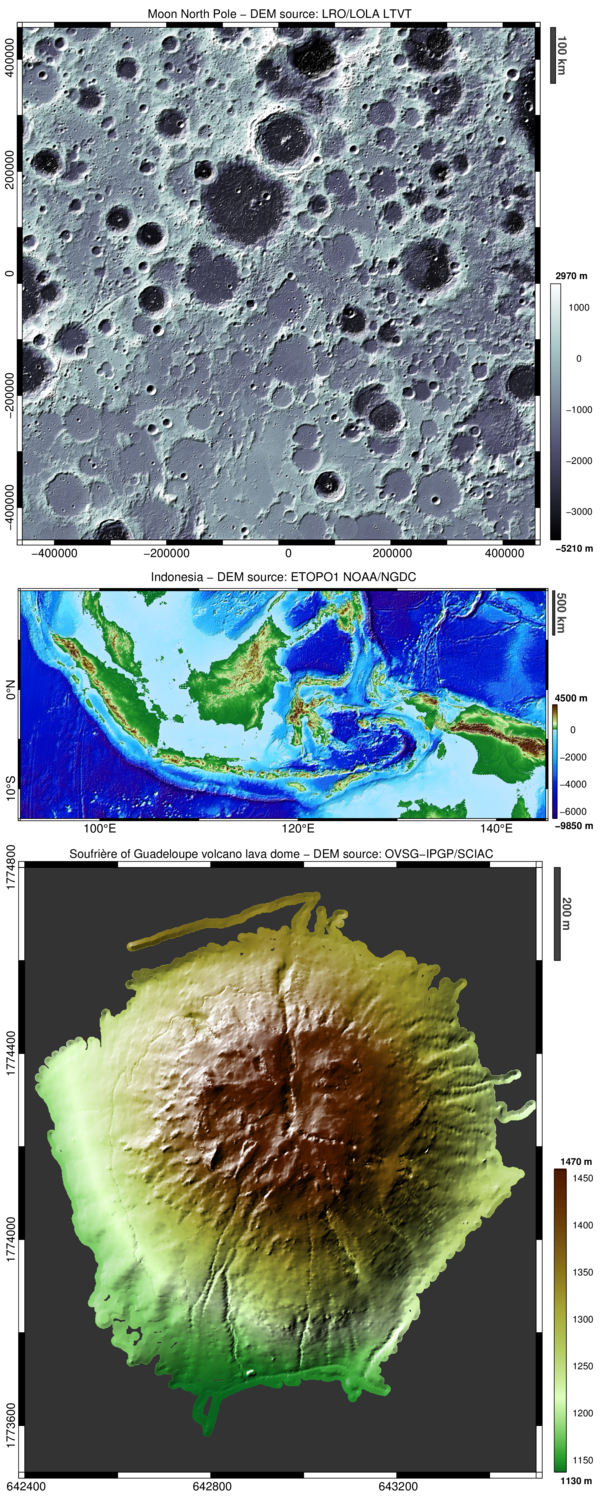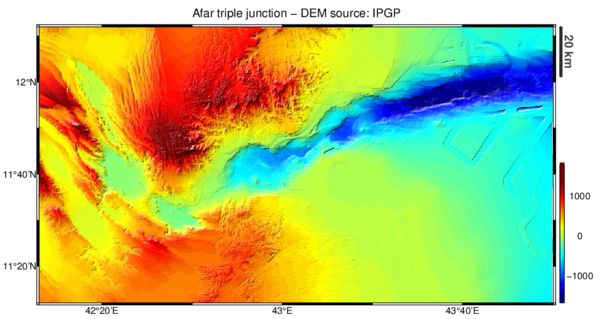This function plots regular grids of elevation X,Y,Z in a more efficient manner than SURFL Matlab's function, because it recomputes lighting and displays result as shaded color flat RGB image. It uses also median-style filter to deal with min/max values of elevations and gradient, and proposes two specific colormaps "landcolor" and "seacolor". Color mapping and lighting parameters can be changed from default values. In addition, several options are available: 'cartesian' to add decimal axis, 'latlon' to add geographical axis (GMT-like), 'legend' for an automatic scaling legend, 'lake' for automatic flat area color-filling and 'interp' to fill the novalue gaps... This may be useful to produce high-quality and moderate-size Postscript image adapted for publication. Figure examples: Moon North Pole using the bone colormap and high contrast lighting (DEM source: raster LRO/LOLA LTVT) Indonesia archipelago using default colormaps, 'dms' axis basemap and legend scales (DEM source: raster NOAA/NGDC ETOPO1) Soufrière of Guadeloupe volcano lava dome: 1-m resolution with NaN values (DEM source: OVSG-IPGP/SCIAC) See "doc dem" for syntax, examples and help. See also the READHGT function from same author. ```DEM Shaded relief image plot DEM(X,Y,Z) plots the Digital Elevation Model defined by X and Y coordinate vectors and elevation matrix Z, as a lighted image using specific "landcolor" and "seacolor" colormaps. DEM uses IMAGESC function which is much faster than SURFL when dealing with large high-resolution DEM. It produces also high-quality and moderate-size Postscript image adapted for publication. [H,I] = DEM(...); returns graphic handle H and illuminated image as I, an MxNx3 matrix (if Z is MxN and DECIM is 1). DEM(X,Y,Z,'Param1',Value1,'Param2',Value2,...) specifies options or parameter/value couple (case insensitive): --- Lighting options --- 'Azimuth',A Light azimuth in degrees clockwise relative to North. Default is A = -45 for a natural northwestern illumination. 'Contrast',C Light contrast, as the exponent of the gradient value: C = 1 for linear contrast (default), C = 0 to remove lighting, C = 0.5 for moderate lighting, C = 2 or more for strong contrast. 'LCut',LC Lighting scale saturation cut with a median-style filter in % of elements, such as LC% of maximum gradient values are ignored: LC = 0.2 is default, LC = 0 for full scale gradient. 'km' Stands that X and Y coordinates are in km instead of m (default). This allows correct lighting. Ignored if LATLON option is used. --- Elevation colorscale options --- 'ZLim',[ZMIN,ZMAX] Fixes min and max elevation values for colormap. Use NaN to keep real min and/or max data values. 'ZCut',ZC Median-style filter to cut extremes values of Z (in % of elements), such that ZC% of most min/max elevation values are ignored in the colormap application: ZC = 0.5 is default, ZC = 0 for full scale. --- "No Value" elevation options --- 'NoValue',NOVALUE Defines the values that will be replaced by NaN. Note that values equal to minimum of Z class are automatically detected as NaN (e.g., -32768 for int16 class). 'NaNColor',[R,G,B] Sets the RGB color for NaN/NoValue pixels (default is a dark gray). Note that your must specify a valid 3-scalar vector (between 0 and 1); color characters like 'w' or 'k' are not allowed, use [1,1,1] or [0,0,0] instead. 'Interp' Interpolates linearly all NaN values (fills the gaps using linear triangulation), using an optimized algorithm. --- Colormap options --- 'LandColor',LMAP Uses LMAP colormap instead of default (landcolor, if exists or jet) for Z > 0 elevations. 'SeaColor',SMAP Sets the colormap used for Z ≤ 0 elevations. Default is seacolor (if exists) or single color [0.7,0.9,1] (a light cyan) to simulate sea color. 'ColorMap',CMAP Uses CMAP colormap for full range of elevations, instead of default land/sea. This option overwrites LANDCOLOR/SEACOLOR options. 'Lake' Detects automaticaly flat areas different from sea level (non-zero elevations) and colors them as lake surfaces. 'Watermark',N Makes the whole image lighter by a factor of N. --- Basemap and scale options --- 'Cartesian' Plots classic basemap-style axis, considering coordinates X and Y as cartesian in meters. Use parameter "km' for X/Y in km. 'LatLon' Plots geographic basemap-style axis in deg/min/sec, considering coordinates X as longitude and Y as latitude. Axis aspect ratio will be adjusted to approximatively preserve distances (this is not a real projection!). This overwrites ZRatio option. 'Legend' Adds legends to the right of graph: elevation scale (colorbar) and a distance scale (in km). 'FontSize',FS Font size for X and Y tick labels. Default is FS = 10. 'BorderWidth',BW Border width of the basemap axis, in % of axis height. Must be used together with CARTESIAN or LATLON options. Default is BW = 1%. --- Decimation options --- For optimization purpose, DEM will automatically decimate data to limit to a total of 1500x1500 pixels images. To avoid this, use following options, but be aware that large grids may require huge computer ressources or induce disk swap or memory errors. 'Decim',N Decimates matrix Z at 1/N times of the original sampling. 'NoDecim' Forces full resolution of Z, no decimation. --- Informations --- Colormaps are Mx3 RGB matrix so it is easy to modify saturation (CMAP.^N), set darker (CMAP/N), lighter (1 - 1/N + CMAP/N), inverse it (flipud(CMAP)), etc... To get free worldwide topographic data (SRTM), see READHGT function. For backward compatibility, the former syntax is still accepted: DEM(X,Y,Z,OPT,CMAP,NOVALUE,SEACOLOR) where OPT = [A,C,LC,ZMIN,ZMAX,ZC], also option aliases DEC, DMS and SCALE, but there is no argument checking. Please prefer the param/value syntax. Author: François Beauducel Created: 2007-05-17 Updated: 2013-03-10 ``` Download dem.m, landcolor.m and seacolor (needed) at Matlab Central file exchange (Copyright 2013 F. Beauducel / BSD License).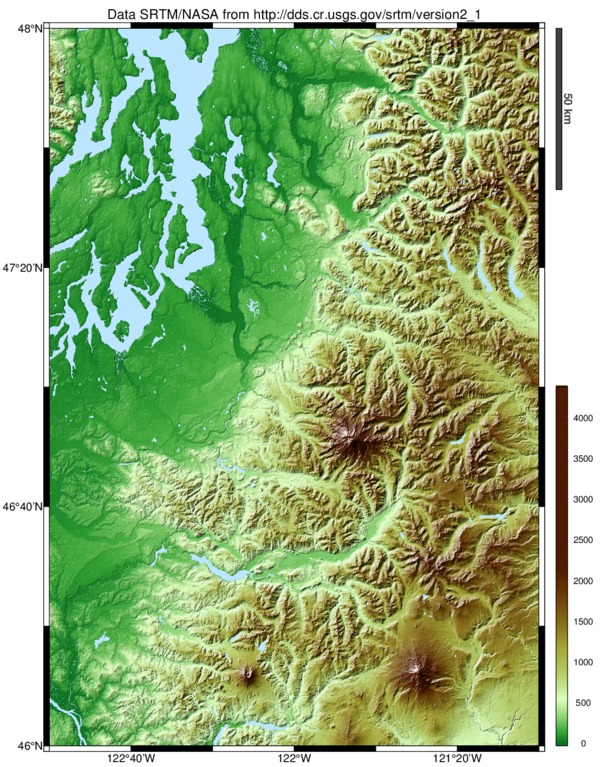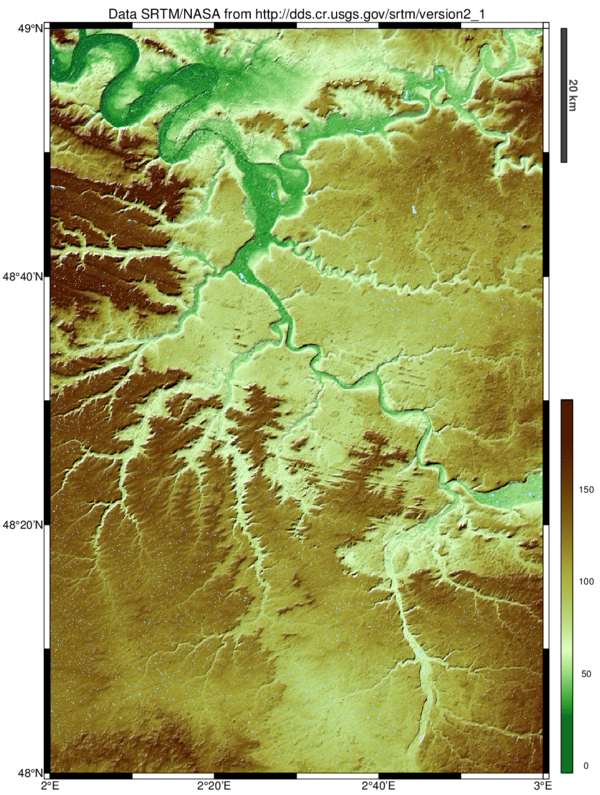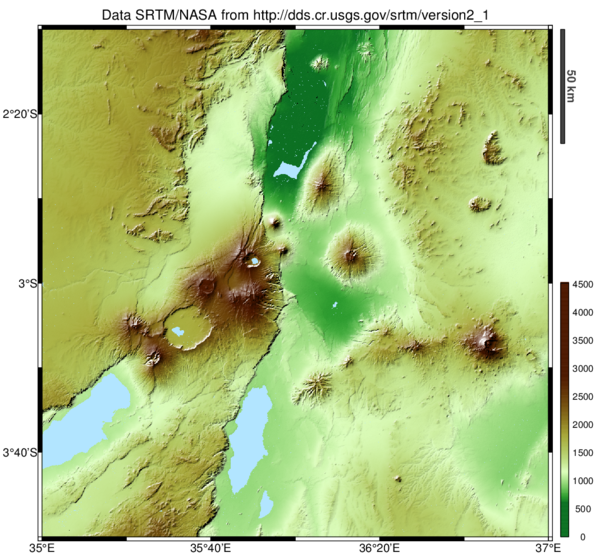This function imports .HGT "height" binary data files from NASA SRTM global digital elevation model of Earth land, corresponding to 1x1 degree tiles of 3-arc seconds resolution (SRTM3, around 90 m) and 1-arc second (SRTM1, around 30 m) for USA territory, and returns coordinates vectors latitude and longitude, and a matrix of elevation values. The function includes an automatic download of data from the USGS SRTM webserver, so indicating latitude and longitude is sufficient to get the data and instant map plot anywhere in the World. Also some basic options as 'merge' to concatenate tiles, 'interp' for gaps (novalue) linear interpolation, 'crop' for rectangle selection inside tile(s). ```READHGT Import/download NASA SRTM data files (.HGT). X=READHGT(FILENAME) reads HGT "height" SRTM data file FILENAME and returns a structure X containing following fields lat: coordinate vector of latitudes (decimal degree) lon: coordinate vector of longitudes (decimal degree) z: matrix of elevations (meters, INT16 class) FILENAME must be in the form "[N|S]yy[E|W]xxx.hgt[.zip]" as downloaded from SRTM data servers. X=READHGT(LAT,LON) attemps to download the file *.hgt.zip corresponding to LAT and LON (in decimal degrees) coordinates (lower-left corner) from the USGS data server (needs an Internet connection and a companion file "readhgt_srtm_index.txt"). Downloaded filename(s) will be given in an additional output structure field X.hgt. LAT and/or LON can be vectors: in that case, tiles corresponding to all possible combinations of LAT and LON values will be downloaded, and optional output structure X will have as much elements as tiles. READGHT(...) without output argument or X=READHGT(...,'plot') plots the tile(s). For better plot results, it is recommended to install DEM personal function available at author's Matlab page. READHGT(LAT,LON,...,'merge'), in case of adjoining values of LAT and LON, will concatenate tiles to produce a single one. ATTENTION: tiles assembling may require huge computer ressources and cause disk swaping or memory error. READHGT(LAT,LON,...,'interp') linearly interpolates missing data. READHGT(...,'crop',[LAT1,lAT2,LON1,LON2]) crops the map using latitude/longitude limits. READHGT(LAT,LON,...,'crop'), without limits argument vector, crops the resulting map around existing land (reduces any sea or novalue areas at the borders). READHGT(LAT,LON,...,'srtm3') forces SRTM3 download (by default, SRTM1 tile is downloaded if exists). Usefull for USA neighborhood. READHGT(LAT,LON,OUTDIR) specifies output directory OUTDIR to write downloaded files. READHGT(LAT,LON,OUTDIR,URL) specifies the URL address to find HGT files (default is USGS). Examples: - to plot a map of the Paris region, France (single tile): readhgt(48,2) - to plot a map of Flores volcanic island, Indonesia (5 tiles): readhgt(-9,119:123,'merge') - to download SRTM1 data of Cascade Range (27 individual tiles): X=readhgt(40:48,-123:-121); Informations: - each file corresponds to a tile of 1x1 degree of a square grid 1201x1201 of elevation values (SRTM3 = 3 arc-seconds), and for USA territory at higher resolution 3601x3601 grid (SRTM1 = 1 arc-second) - elevations are of class INT16: sea level values are 0, unknown values equal -32768 (there is no NaN for INT class), use 'interp' option to fill the gaps - note that borders are included in each tile, so to concatenate tiles you must remove one row/column in the corresponding direction (this is made automatically with the 'merge' option) - downloaded file is written in the current directory or optional OUTDIR directory, and it remains there - NASA Shuttle Radar Topography Mission [February 11 to 22, 2000] produced a near-global covering on Earth land, but still limited to latitudes from 60S to 60N. Offshore tiles will be output as flat 0 value grid - if you look for other global topographic data, take a look to ASTER GDEM, worldwide 1 arc-second resolution (from 83S to 83N): http://gdem.ersdac.jspacesystems.or.jp (free registration required) Author: François Beauducel Institut de Physique du Globe de Paris References: http://dds.cr.usgs.gov/srtm/version2_1 Acknowledgments: Yves Gaudemer Created: 2012-04-22 Updated: 2013-01-17 ``` Download readhgt.m and readhgt_srtm_index.txt (needed) at Matlab Central file exchange (Copyright 2012 F. Beauducel / BSD License).

## LL2UTM and UTM2LL: Latitude/longitude to and from UTM coordinates precise and vectorized conversion.

 UTM2LL converts Universal Transverse Mercator (UTM) East/North coordinates to latitude/longitude. LL2UTM converts latitude/longitude coordinates to UTM. Both functions are using precise formula (millimeter precision), possible user-defined datum (WGS84 is the default), and are all vectorized (no loop in the code). It means that huge matrix of points, like an entire DEM grid, can be converted very fast. Example (needs readhgt.m author's function): ```X = readhgt(36:38,12:15,'merge','crop',[36.5,38.5,12.2,16],'plot'); [lon,lat] = meshgrid(X.lon,X.lat); [x,y,zone] = ll2utm(lat,lon); z = double(X.z); z(z==-32768 | z<0) = NaN; figure pcolor(x,y,z); shading flat; hold on contour(x,y,z,[0,0],'w') hold off; axis equal; axis tight xlabel('East (m)'); ylabel('North (m)') title(sprintf('Sicily - UTM zone %d WGS84',zone)) ``` loads SRTM full resolution DEM of Sicily in lat/lon (a 2400x4500 grid), converts it to UTM and plots the result. To make a regular UTM grid, you may interpolate x and y with griddata function. See "doc ll2utm" and "doc utm2ll" for syntax and help. ```LL2UTM Lat/Lon to UTM coordinates precise conversion. [X,Y]=LL2UTM2(LAT,LON) converts coordinates LAT,LON (in degrees) to UTM X and Y (in meters). Default datum is WGS84. LAT and LON can be scalars, vectors or matrix. Outputs X and Y will have the same size as inputs. LL2UTM(LAT,LON,DATUM) uses specific DATUM for conversion. DATUM can be a string in the following list: 'wgs84': World Geodetic System 1984 (default) 'nad27': North American Datum 1927 'clk66': Clarke 1866 'nad83': North American Datum 1983 'grs80': Geodetic Reference System 1980 'int24': International 1924 / Hayford 1909 or DATUM can be a 2-element vector [A,F] where A is semimajor axis (in meters) and F is flattening of the user-defined ellipsoid. [X,Y,ZONE]=LL2UTM(...) returns also computed UTM ZONE (negative value stands for southern hemisphere points). Notice: - LL2UTM does not perform cross-datum conversion. - precision is near a millimeter. Reference: I.G.N., Projection cartographique Mercator Transverse: Algorithmes, Notes Techniques NT/G 76, janvier 1995. Author: François Beauducel Institut de Physique du Globe de Paris Created: 2003-12-02 Updated: 2014-02-17 ``` ```UTM2LL UTM to Lat/Lon coordinates precise conversion. [LAT,LON]=UTM2LL(X,Y,ZONE) converts UTM coordinates X,Y (in meters) defined in the UTM ZONE (integer) to latitude LAT and longitude LON (in degrees). Default datum is WGS84. X and Y can be scalars, vectors or matrix. Outputs LAT and LON will have the same size as inputs. For southern hemisphere points, use negative zone -ZONE. UTM2LL(X,Y,ZONE,DATUM) uses specific DATUM for conversion. DATUM can be a string in the following list: 'wgs84': World Geodetic System 1984 (default) 'nad27': North American Datum 1927 'clk66': Clarke 1866 'nad83': North American Datum 1983 'grs80': Geodetic Reference System 1980 'int24': International 1924 / Hayford 1909 or DATUM can be a 2-element vector [A,F] where A is semimajor axis (in meters) and F is flattening of the user-defined ellipsoid. Notice: - UTM2LL does not perform cross-datum conversion. - precision is near a millimeter. Reference: I.G.N., Projection cartographique Mercator Transverse: Algorithmes, Notes Techniques NT/G 76, janvier 1995. Author: Francois Beauducel, Created: 2001-08-23 Updated: 2014-02-17 ``` Download ll2utm.m and utm2ll.m at Matlab Central file exchange (Copyright 2014 F. Beauducel / BSD License).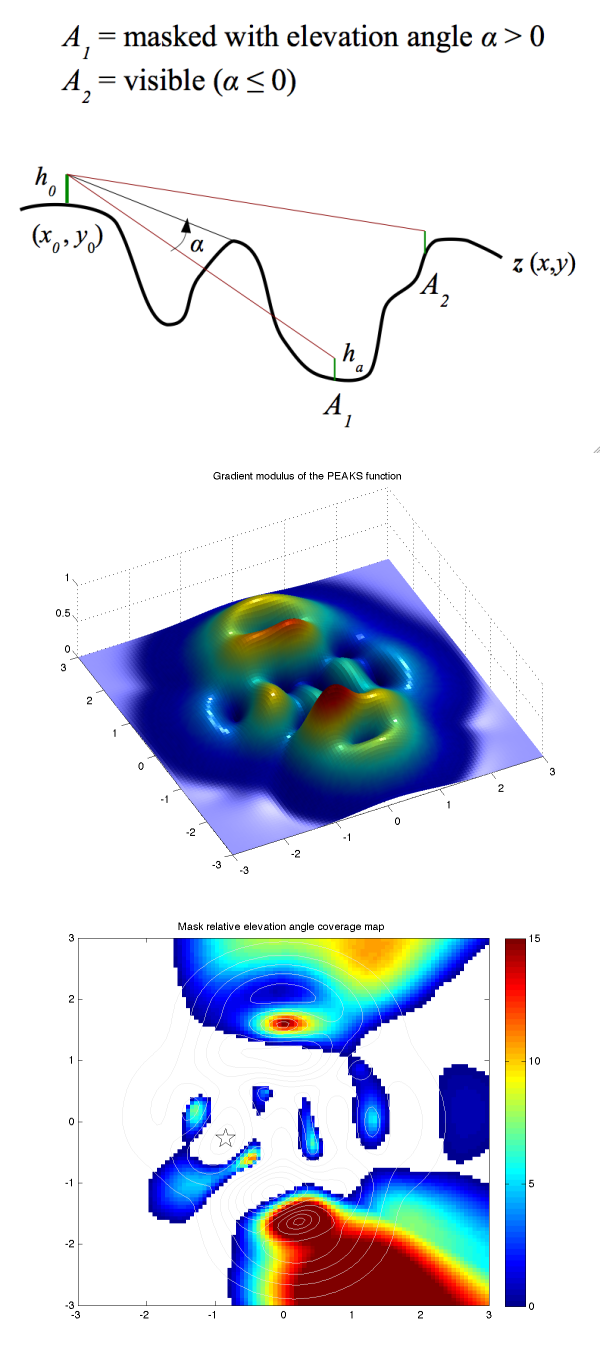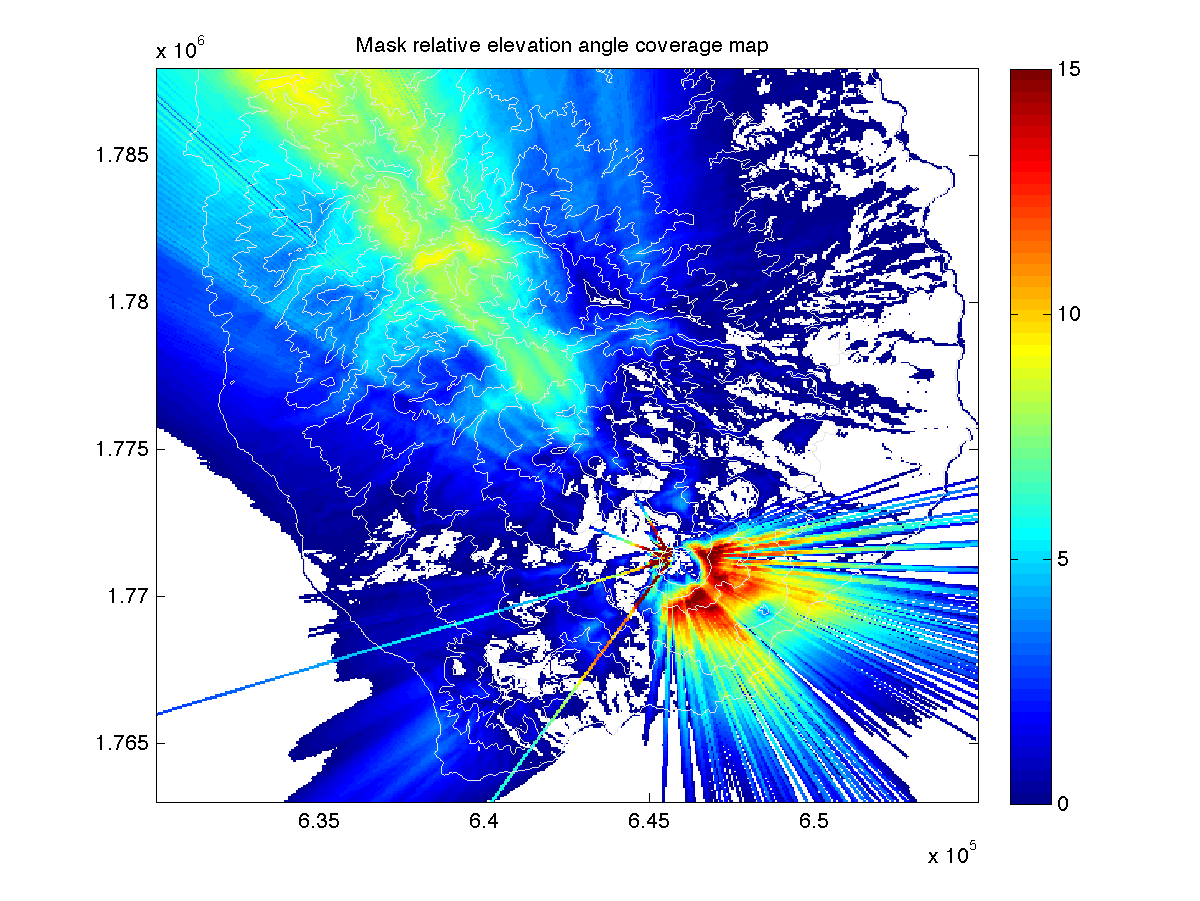This program computes a map of visibility from a selected point on a topography. It has been written to help the search for radio relay best location. Because it considers only direct line of sight, it gives a good estimation for possible radio link for short distances only (less than 10 km), neglecting curvature of the Earth, Fresnel zone and atmospheric refraction on radio waves propagation. The program computes the relative elevation angle of the mask for each point (the angle is null or negative if the point is visible). The function needs a digital elevation model Z and associated (X,Y) vectors or matrices of coordinates (same unit as Z), position of the point (X0,Y0), the antenna height H0 (for instance 4 m), and the hypothetic antenna height Ha on each topography points (for instance 3 m). When no output argument is given, the function plots a map of the results (color map of mask angles, and blank for visible points, see example screenshot). The script is not fully optimized because it makes a global loop on the matrix elements to compute each profile of topography... (I didn't find (yet) a way to fully vectorize the problem), so it takes some time to compute, depending on the number of element of Z. However, I found a faster algorithm (about 2 times faster), giving approximate result but usefull to process a first map. See help for syntax, and script comments for details. ```RADIOCOVER Radio link coverage map. RADIOCOVER(X,Y,Z,X0,Y0,H0,Ha) computes the coverage map of possible direct linear radio link from the point (X0,Y0) with antenna height H0, using digital terrain model defined by coordinate vectors X and Y, and elevation matrix Z, and hypothetic antenna height Ha, then plots a color map of the relative elevation mask angle (in degrees) with blank areas where there is no mask (visible), together with a contour map of the topography. X and Y can be vectors with length(X) = n and length(Y) = m where [m,n] = size(Z), or matrices of the same size as Z (as from MESHGRID). RADIOCOVER(...,METHOD) specifies alternate methods. The default is nearest neighbor interpolation. Available methods are: 'nearest' - nearest neighbor interpolation (default) 'linear' - linear interpolation (smoother result) 'fast' - approximate algorithm (about 2 times faster) M = RADIOCOVER(...); returns a matrix of relative elevation mask angle (in degrees, same size as Z), without producing graphic. Visible points have null or negative values. The model assumes linear propagation of radio waves (direct line of sight between the two antennas), and neglects curvature of the Earth, Fresnel zone, and atmospheric refraction. Example: [x,y,z]=peaks(100); [fx,fy]=gradient(z); z=sqrt(fx.^2+fy.^2); surf(x,y,z), shading flat, light, view(-24,74) radiocover(x,y,z,-0.84,-0.27,.05,.05) Author: François Beauducel Institut de Physique du Globe de Paris Created: 2003-01-10 Modified: 2010-01-08 ``` Download radiocover.m at Matlab Central file exchange (Copyright 2003-2009 F. Beauducel / BSD License).

## GREATCIRCLE and LOXODROME: shortest and rhumb line path, distance and bearing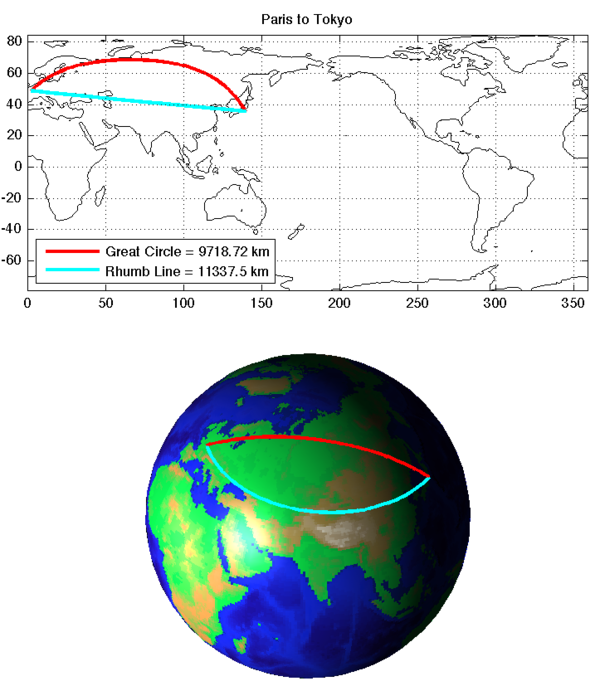The function GREATCIRCLE computes the shortest path along the great circle ("as the crow flies") between two points defined by their geographic coordinates (latitude and longitude). With one output argument it returns distance or vector of distances, with two or more output arguments it returns path coordinates and optional vector of distances and bearing angles along the path. The function LOXODROME computes the path with a constant bearing, crossing all meridians of longitude at the same angle. It returns also a vector of distances and the bearing angle. Loxodrome path (also known as "rhumb line") is longer than great circle one, but still used in navigation as it is easier to follow with a compass. Type 'doc greatcircle' or 'doc loxodrome' for syntax, help and examples. ```GREATCIRCLE "As the crow flies" path, distance and bearing. GREATCIRCLE(LAT1,LON1,LAT2,LON2) returns the shortest distance (in km) along the great circle between two points defined by spherical coordinates latitude and longitude (in decimal degrees). If input arguments are vectors or matrix, it returns a vector/matrix of distances of the same size. [LAT,LON,DISTANCE,BEARING]=GREATCIRCLE(LAT1,LON1,LAT2,LON2) where all input arguments are scalars, computes the way points of coordinates LAT and LON, the distances (in km), and the bearing angles (in degrees from North) along the path, with default 100 intermediate points. Note that last bearing angle is NaN. [...]=GREATCIRCLE(...,N) uses N intermediate points. Example: load topo contour(0:359,-89:90,topo,[0,0],'k') [lat,lon,dis] = greatcircle(48.8,2.3,35.7,139.7); hold on, plot(lon,lat,'r','linewidth',2), hold off title(sprintf('Paris to Tokyo = %g km',dis(end))) See also LOXODROME. Author: Francois Beauducel Created: 2012-07-26 Updated: 2012-11-14 References: http://www.movable-type.co.uk/scripts/latlong.html http://en.wikipedia.org/wiki/Haversine_formula ``` ```LOXODROME Rhumb line path and distance. [LAT,LON]=LOXODROME(LAT1,LON1,LAT2,LON2) computes the line between two points defined by their spherical coordinates latitude and longitude (in decimal degrees), crossing all meridians of longitude at the same angle, i.e. a path derived from an initial and constant bearing. LAT and LON contain vectors of coordinates of the way points. [LAT,LON,DISTANCE,BEARING]=LOXODROME(...) returns also vector of distances (in km) along the path way, and constant bearing angle (in degrees from North, a scalar). [...]=LOXODROME(...,N) uses N intermediate points (default is 100). Example: load topo contour(0:359,-89:90,topo,[0,0],'k') [lat,lon,dis,bear] = loxodrome(48.8,2.3,35.7,139.7); hold on, plot(lon,lat,'r','linewidth',2), hold off title(sprintf('Paris to Tokyo = %g km - bear = %g N',dis(end),bear)) See also GREATCIRCLE. Author: Francois Beauducel Created: 2012-10-30 Updated: 2012-11-08 References: http://en.wikipedia.org/wiki/Rhumb_line Acknowledgments: Jorge David Taramona Perea, Jacques Vernin. ``` Download greatcircle.m and loxodrome.m at Matlab Central file exchange (Copyright 2012 F. Beauducel / BSD License).

## COMPROSE: Compass rose plot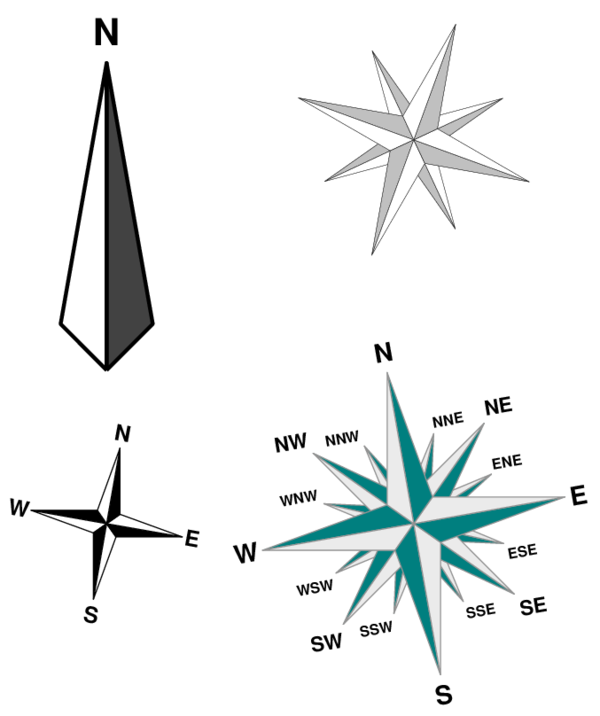This little function draws a compass rose with optional Cardinal directions with basic properties: 1, 4, 8, or 16-points position X,Y on current axis size (in axis unit) azimuth (in degrees) accepts any additional patch param/value pairs, like 'FaceColor', 'EdgeColor', 'LineWidth', ... displays Cardinal point names (optional) Type 'doc comprose' for syntax, help and examples. ```COMPROSE Compass rose plot COMPROSE(X,Y,N,W,AZ) adds a compass rose on current axis located at position X,Y with N points (N is 1, 4, 8 or 16), width W (radius) and North pointing to azimuth AZ (in degree, AZ = 0 means an arrow pointing to positive Y-axis direction, rotating clockwise). COMPROSE(...,FS) adds Cardinal directions with font size FS. COMPROSE(...,'param1',value1,'param2',value2,...) specifies any additionnal properties of the Patch using standard parameter/value pairs. Note that 'FaceColor' concerns only the default black-filled parts of the drawing. H=COMPROSE(...) returns a Nx3 matrix of object handles: first column addresses solid filled patches, second column for white patches, third column for Cardinal direction text. Examples: comprose(0,0,8,.5,10) comprose(2,-1,1,2,0,20,'LineWidth',2,'FaceColor',.5*[1,1,1]) h = comprose(1,2.5,16,1,-10); set(h(:,1),'FaceColor',[0,.5,.5],'EdgeColor',.5*[1,1,1]) set(h(:,2),'FaceColor',.9*[1,1,1]) See also PATCH. Author: Francois Beauducel Created: 2012-06-24 ``` Download comprose.m at Matlab Central file exchange (Copyright 2012 F. Beauducel / BSD License).

## ARROWS: generalized 2-D arrows plot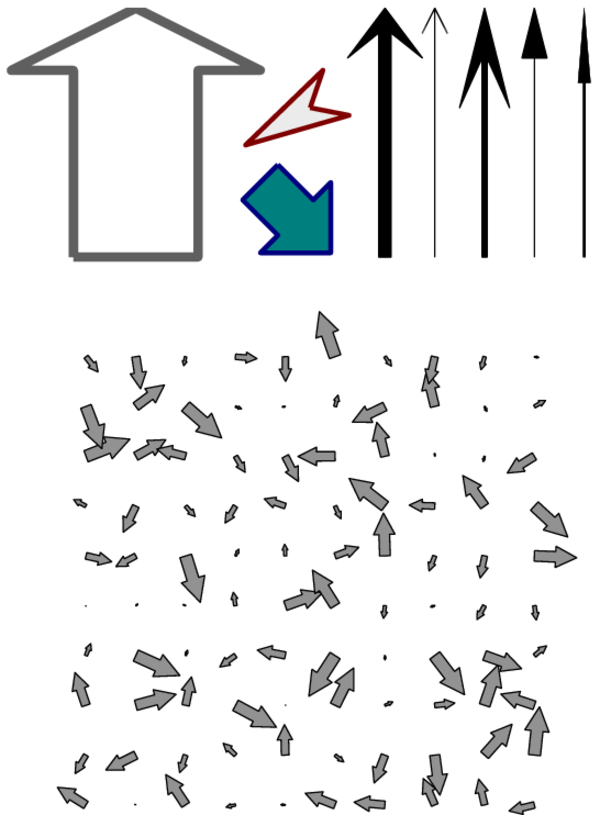This little function could be an alternative to other existing arrow plot functions, since it has very simple, vectorized and effective coding. In fact, arrows are all plotted in a single patch command! The function also accepts any standard patch parameter/value pair like 'FaceColor', 'EdgeColor', 'LineWidth', etc. It can be used to plot a personalized arrow at coordinates X,Y with length L and azimuth AZ, or any of these arguments can be vector or matrix, like QUIVER function, to plot multiple arrows. Arrow's aspect ratio, head and segment line shapes are configurable with 4 parameters: head width, head length, head inside length and segment line width, all normalized to arrow's length. Type 'doc arrows' for syntax, help and examples. ```ARROWS Generalised 2-D arrows plot ARROWS(X,Y,L,AZ) draws an arrow on current axis from position X,Y with length L, oriented with azimuth AZ (in degree, AZ = 0 means an arrow pointing to positive Y-axis direction, rotating clockwise). X and Y can be scalars or matrix. In the last case, any or both L and AZ can be scalars or matrix of the same size as X and Y. ARROWS(...,SHAPE) uses relative ratios SHAPE = [HEADW,HEADL,HEADI,LINEW] to adjust head width HEADW, head length HEADL, head inside length HEADI, and segment line width LINEW for an arrow length of 1 (default is SHAPE = [1,0.25,0.25,0.5]). ARROWS(...,'param1',value1,'param2',value2,...) specifies any additionnal properties of the Patch using standard parameter/value pairs, like 'FaceColor','EdgeColor','LineWidth', ... H=ARROWS(...) returns graphic's handle of patches. Examples: arrows(0,0,1,45,'FaceColor','none','LineWidth',3) arrows(1,0,1,0,[.2,.4,.2,.02]) [xx,yy] = meshgrid(1:10); arrows(xx,yy,rand(size(xx)),360*rand(size(xx))) Notes: Arrow shape supposes an equal aspect ratio (axis equal). Equivalent of quiver(x,y,u,v,0) command is obtained with: [th,l] = cart2pol(u,v); arrows(x,y,l,90 - th*180/pi) See also PATCH, QUIVER. Author: Francois Beauducel Created: 1995-02-03 Updated: 2012-06-30 ``` Download arrows.m at Matlab Central file exchange (Copyright 2012 F. Beauducel / BSD License).

## DOODSON: Doodson tidal wave components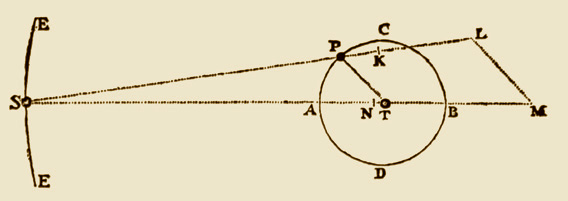This function gives the parameters for about 40 main wave components of Earth tides due to Sun and Moon (annual, semiannual, monthly, fortnightly, diurnal, semidiurnal, ...) using the harmonic development of Arthur Thomas Doodson [1890-1968]. For a given wave, it returns in a structure the 6 Doodson's arguments, wave Darwin's symbol, wave description and the wave period (in days). Example:```>> X=doodson('M2') X = symbol: {'M2'} name: {'Principal lunar semidiurnal'} doodson: [2 0 0 0 0 0] period: 0.5175 ``` Type doodson without argument to see the list of available waves. ```DOODSON Doodson tidal wave components X = DOODSON(WAVE) returns a structure containing, for the tidal wave symbol WAVE, the following fields: doodson: vector of the 6 Doodson arguments symbol: wave symbol string (Darwin's notation) name: wave long name period: wave period (in days) X = DOODSON returns all known waves parameters. Type DOODSON without any input/output argument displays a table of available waves. References: Agnew D.C. (2007), Earth Tides, in « Treatise on Geophysics: Geodesy », T. A. Herring Ed., Elsevier, New York. Author: Francois Beauducel Created: 2014-05-22 Updated: 2014-05-24 ``` Download doodson.m at Matlab Central file exchange (Copyright 2014 F. Beauducel / BSD License).

## READIS2: Import IS2 files (Fluke infrared camera)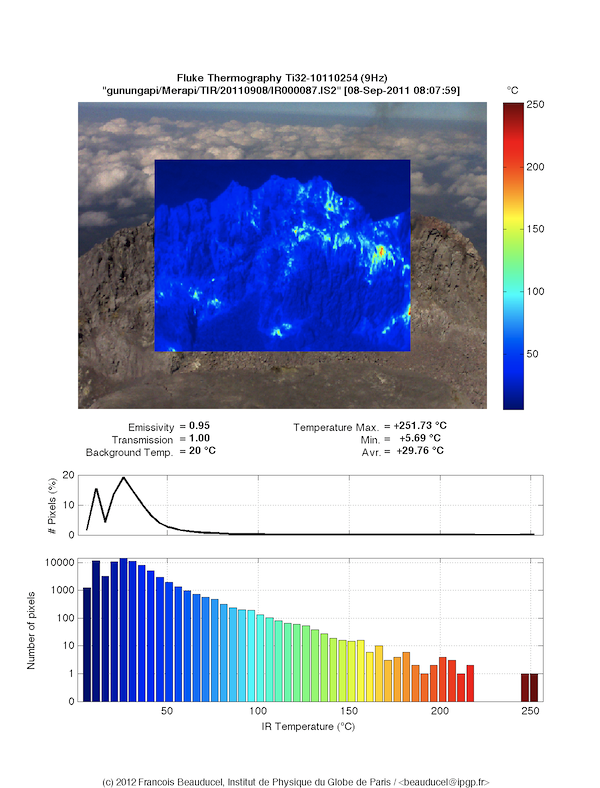This function reads data files from Fluke TI32 infrared thermal camera (.IS2 proprietary format). It returns data images (raw and calibrated temperatures, and visible RGB image) and some of the header information in a single structure. Without output arguments, it plots blended infrared and visible images and a histogram of temperatures. The function has been tested for TI32 camera original files only. Presently it does not work with files edited with the Fluke software, neither for other cameras. Also the function needs a separated calibration file since I was not able to find the original tables in the binary file (but I'm quite sure they exist...). Any help to improve that is welcome. ```READIS2 Import data files from Fluke infrared thermal camera (IS2 format). X=READIS2(FILENAME) reads IS2 radiometric file FILENAME and returns a structure X containing fields with data and headers: IR: infrared sensor values (240x320 uint16 matrix) TB: brillancy temperatures, in °C (240x320 double matrix) T: body temperatures, in °C (240x320 double matrix) VI: visible image (480x640x3 uint8 RGB) VI2: cropped and interpolated visible image adjusted on IR image pixels (240x320x3 uint8 RGB) Emissivity: emissivity value (0 to 1.00) Transmission: transmission value (0 to 1.00) BackgroundTemp: background temperature (°C) Date: [YEAR MONTH DAY HOUR MINUTE SECOND] X=READIS2(FILENAME,MAP) plots temperature image using MAP color scale over visible image. MAP is a valid [n x 3] RGB colormap, for instance JET or GRAY. Use MAP=[] to specify other parameters without plot. X=READIS2(FILENAME,MAP,PARAM) applies given parameters to overwrite values from camera. PARAM = [DT,EM,TR,BT] (use NaN to keep original values): DT = time difference (in days) applied to image timestamp EM = emissivity TR = transmission BT = background temperature (°C) X=READIS2(FILENAME,MAP,PARAM,CAL) applies a polynomial function to calibrate brillancy temperatures, as follow: TB_cal = polyval(CAL,TB_raw). This will affect also body temperatures. Example: CAL = [1.08,-2.72] is for my own Ti32 camera calibration result for 0-100°C range laboratory experiment. Notes: - the function has been tested only with original IS2 files from a Fluke TI32 camera. - to produce temperatures from IR sensor values, the function needs a calibration file 'readis2_ti32.dat' located in current directory or Matlab path (see corresponding header info). - body temperatures Tr (output X.T) and brillancy temperatures Tb (output X.IR) are related with the formula: Tb = ε*τ*Tr + (2-ε-τ)*Te where ε is emissivity, τ is transmission, and Te is background (reflected) temperature. So Tb and Tr are equal for ε = τ = 1. Author: François Beauducel Institut de Physique du Globe de Paris Created: 2011-07-24 Updated: 2012-04-01 Acknowledgments: Magnus Andersen (read_is2.m), Pierre Agrinier, Valérie Clouard, Damien Gaudin, David Krammer. ``` Download readis2.m and readis2_ti32.dat (needed) at Matlab Central file exchange (Copyright 2012 F. Beauducel / BSD License).

## POLARMAP: Polarized color mapWhen representing "polarized" data for which zero and sign are meaningful (like for instance, a gravity anomalies map), it is useful to have a colormap where white stands for zero value, and greater magnitudes are plotted as colors with increasing saturation. This little function POLARMAP has two different usages: proposes a new red-white-blue colormap that is standard for this purpose (same usage as other Matlab colormaps); applies a zero-center white shading to any existing colormap, like JET or HSV, or user defined. ```POLARMAP Polarized color map POLARMAP applies a "polarized" red-white-blue colormap to current figure, and adjusts the color axis limits to be centered to zero. POLARMAP(M) fixes the number of colors to M (default is 64). POLARMAP(MAP) applies linear shading to white to the center of colormap MAP which can be any of existing colormaps (an Mx3 matrix of RGB). POLARMAP(MAP,C) uses exponent C to modify the shading contrast. Default is C = 1 for linear shading. Use C = 2 to strengthen the shading, or C = 0.5 to attenuate it. C=POLARMAP(...) returns an M-by-3 matrix containing the colormap, that can be used with COLORMAP function like other colormaps. Examples: pcolor(peaks), shading interp polarmap, colorbar then try the following polarmap(jet,0.5) Note the polar shading has no real interest with colormaps that include white color as one of the extremes (like GRAY, BONE, HOT, ...). See also JET, HSV, COPPER, SPRING, SUMMER, WINTER, COOL, COLORMAP, RGBPLOT. Author: François Beauducel Created: 2011-10-26 Updated: 2012-06-09 ``` Download polarmap.m at Matlab Central file exchange (Copyright 2012 F. Beauducel / BSD License).

## MOVSUM: Unbiased histogram method (moving sum)This function is a simple digital filter with one's coefficients, nearly equivalent to moving average but instead of mean values, it computes the sum. This is particularly usefull for rainfall plots for which it is, in my opinion, the only good way to show the data. The figure attempts to demonstrate it. Let us have some rainfall data at 10-minute sampling interval, and simply want to plot the 1-hour rainfall... If you use classical histogram representation, it leads to artifacts that depends on a priori time windows offset you chose (see figure): histogram #1 based on rounded hour intervals, shows two 6-mm values between 02:00 and 04:00, and totally misses the 11-mm maximum at 03:30, histogram #2 is the same 1-hour histogram but shifted by 20 minutes; it seems better but does not show the 5-mm maximum at 1:30, histogram #3 is shifted by 40-minutes and differs again from the first two, As you can see, only the "moving sum" curve shows real 1-hour rainfall values at each time. ```MOVSUM Moving sum plot. Y = MOVSUM(X,N) returns the "moving sum" of X on N consecutive values. Y has the same size as X, and each element of Y is the sum of the N previous values of X. If X is a regularly spaced data vector, then Y corresponds to a digital filter with N b-coefficient equal to one: y(n) = x(n) + x(n-1) + ... + x(1) This is nearly equivalent to a moving average, but instead of mean it is the sum. If X is a matrix, MOVSUM works down the columns. A typical use is for rainfall data vectors. For instance, if X contains rainfall values at 1-minute interval, MOVSUM(X,60) will be the hourly rainfall, expressed as a continous vector of the same size as X. Compared to classical histogram representation, this method avoids some artifacts due to windowing using a priori time intervals. MOVSUM(...) without output arguments produces a plot of the results. Author: François Beauducel Created: 2005 Updated: 2010-10-10 ``` Download movsum.m (Copyright 2010 F. Beauducel / BSD License).

## ROUNDSD: Round with fixed significant digits

 This little function rounds a number (or the elements of a vector or matrix) towards the nearest number with N significant digits. This is a useful complement to Matlab's ROUND, ROUND10 and ROUNDN (Mapping toolbox), especially when dealing with data with a large variety of order of magnitudes. ```ROUNDSD Round with fixed significant digits ROUNDSD(X,N) rounds the elements of X towards the nearest number with N significant digits. ROUNDSD(X,N,METHOD) uses following methods for rounding: 'round' - nearest (default) 'floor' - towards minus infinity 'ceil' - towards infinity 'fix' - towards zero Examples: roundsd(0.012345,3) returns 0.0123 roundsd(12345,2) returns 12000 roundsd(12.345,4,'ceil') returns 12.35 See also Matlab's functions ROUND, ROUND10, FLOOR, CEIL, FIX, and ROUNDN (Mapping Toolbox). Author: François Beauducel Institut de Physique du Globe de Paris Acknowledgments: Edward Zechmann, Daniel Armyr, Yuri Kotliarov Created: 2009-01-16 Updated: 2015-04-03 ``` Download roundsd.m at Matlab Central file exchange (Copyright 2015 F. Beauducel / BSD License). NEWS: This file was selected as MATLAB Central Pick of the Week, and integrated as an option of the core function ROUND in the new Matlab 2014b !

## MINMAX: Generalized median-style filterA little function that I imagined many years ago: a generalized "median-style" filter, an elegant way to generalize MIN, MAX, MEDIAN and extreme filtering functions, reduced to a single parameter. Let's sorting all values of a vector X in ascending order, thus consider the normalized rank position N of elements: N = 0 (first value) corresponds to the minimum of X; N = 1 (last value) is the maximum; N = 0.5 (middle position) is the median value; So what is N = 0.9 ? Answer is: it's the maximum of X after excluding the 10% amount of highest values. A typical example of use is minmax(X,[0.01 0.99]) which returns minimum and maximum values of X(:) but excluding the 1% amount of extreme values. This is particularily efficient for automatic scaling of noisy data (see the screenshot example), compared to the use of MEAN and STD functions which is biased by any high-magnitude values in X. Default behavior of MINMAX is to return a vector of minimum and maximum values. So minmax(X) is an abreviation of minmax(X,[0 1]), and equivalent of [min(X(:)) max(X(:))]. Compared to the built-in functions MIN and MAX, it does not return a vector from matrix but returns a scalar by considering all elements of matrix X. Also, it removes any NaN values (returns NaN only if all X values are NaN). Type 'doc minmax' for syntax, help and other examples. ```MINMAX Generalized median filter. Y=MINMAX(X) returns a 2 element vector Y = [min(X) max(X)]. Minimum and maximum values of X(:) are computed after excluding any NaN values. Y=MINMAX(X,N) with N between 0 and 1, returns generalized median filter value of X(:), e.g., it sorts elements of X then interpolates at (100*N) % rank position. N can be scalar, vector or matrix, so Y will have the same size as N. Examples: MINMAX(X,0) returns the minimum value of X elements. MINMAX(X,1) returns the maximum value of X elements. MINMAX(X,0.5) returns the median value of X elements. This is the equivalent of MEDIAN(X(:)). MINMAX(X,0.9) returns maximum value of X after excluding the 10% highest value elements. MINMAX(X,[0 1]) is the same as MINMAX(X). MINMAX(X,[0.01 0.99]) is a convenient way to compute automatic scale of X when samples are noisy, since it filters the 1% elements with extreme values. It may be used for color scaling with CAXIS or for plot scaling with set(gca,'YLim',...). See also MIN, MAX and MEDIAN. Author: François Beauducel Created: 1996 Updated: 2012-10-10 ``` Download minmax.m at Matlab Central file exchange (Copyright 2012 F. Beauducel / BSD License).

## HARMFIT: Sinusoidal harmonic curve fitting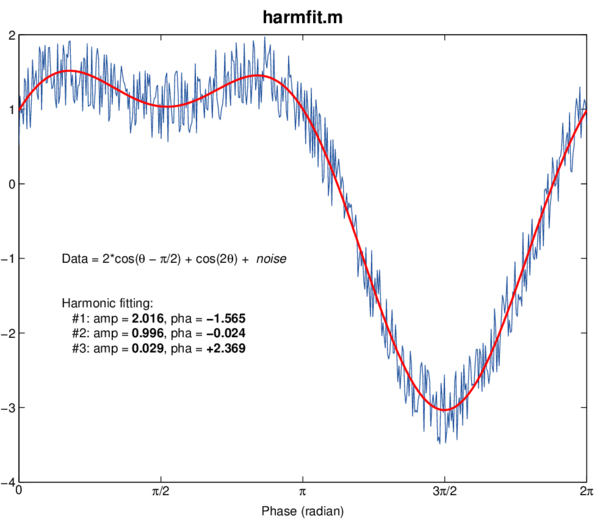This is the core formula of discrete Fourier transform: it simply computes the amplitude and phase shift of fundamental or harmonics of a phase signal. Example:``` t = linspace(0,2*pi); x = 2*cos(t + pi/2) - cos(3*t) + rand(size(t)); harmfit(t,x,1:4) ``` returns estimations of amplitudes/phases for the first four harmonics. ```HARMFIT Sinusoidal harmonic curve fitting. H = HARMFIT(X,Y) returns first harmonic amplitude and phase of the data vector Y relative to phase vector X (in radian) in a 3-element vector H = [N,AMP,PHA], so that AMP*COS(N*X + PHA) fits Y. HARMFIT(X,Y,N) computes the N'th harmonic. N = 1 stands for fundamental, N = 2 is second harmonic, etc... N can be a scalar or a vector of positive integers. For example use N = 1:4 to compute the first four harmonics. [H,YY] = HARMFIT(...) returns also an evaluation of harmonic curve fit in vector YY (as the sum of harmonics in N). This is simply the core calculation of discrete Fourier transform. Example: t = linspace(0,2*pi); x = 2*cos(t + pi/2) - cos(3*t) + rand(size(t)); harmfit(t,x,1:4) returns estimations of amplitudes/phases for the first four harmonics. Note that negative amplitudes are fitted with positive value with a PI phase difference. Author: François Beauducel Institut de Physique du Globe de Paris Created: 2014-05-22 Updated: 2014-05-24 ``` Download harmfit.m at Matlab Central file exchange (Copyright 2014 F. Beauducel / BSD License).

## ROMAN2NUM and NUM2ROMAN: modern Roman numerals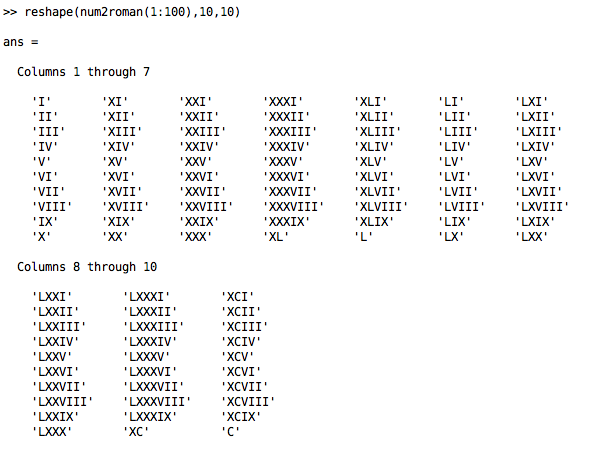These two scripts convert Roman numerals to and from any integer (scalar, vector or matrix), including large numbers greater than 4999 with the parenthesis notation (multiplies by 1000). The function NUM2ROMAN uses strict rules of modern notation (substractive principle for 4 and 9 bases) except for the common 'MMMM' form replacing '(IV)'. ROMAN2NUM is more flexible and is able to convert some other Roman notation possibilities, for instance the 3 different expressions roman2num({'IC','XCIX','XCVIIII'}) return [99,99,99], or roman2num({'MDXV','MCCCCCXV'}) return [1515,1515]. See help for syntax, and script comments for details. ```NUM2ROMAN Roman numerals. NUM2ROMAN(N) returns modern Roman numeral form of integer N, which can be scalar (returns a string), vector or matrix (returns a cell array of strings, same size as N). The function uses strict rules whith substractive notation and commonly found 'MMMM' form for 4000. It includes also parenthesis notation for large numbers (multiplication by 1000). Examples: num2roman(1968) num2roman(10.^(0:7)) reshape(num2roman(1:100),10,10) See also ROMAN2NUM. Author: François Beauducel Institut de Physique du Globe de Paris Created: 2005 Modified: 2009-12-21 ``` ```ROMAN2NUM Roman numerals conversion. ROMAN2NUM(X) converts to scalar the string X (or cell array of strings) of modern Roman numerals. The function considers strict rules with parenthesis notation for numbers larger than 4999. Invalid characters will produce NaN. Example: num2roman('MMMMDCCCLXXXVIII') num2roman('(CCCXIV)CLIX') See also NUM2ROMAN. Author: François Beauducel Institut de Physique du Globe de Paris Created: 2009-12-19 Modified: 2009-12-21 ``` Download num2roman.m and roman2num.m at Matlab Central file exchange (Copyright 2005-2009 F. Beauducel / BSD License).

## EASTER: Easter dayThis little function computes the date of Easter Sunday, using Oudin's algorithm for Julian calendar (starting 325 AD) and Gregorian calendar (after 1583 AD). Easter day is usefull to calculate other christian feasts, like Ash Wednesday (Easter - 46), Good Friday (Easter - 2), Ascension Thursday (Easter + 39), Pentecost (Easter + 49). Some translations of Easter: Pâques (French), Pascua (Spanish), Ostern (German), Pasqua (Italian), Paskah (Indonesian), Wielkanoc (Polish) ... See help for syntax, and script comments for details. ```EASTER Easter Day EASTER displays the date of Easter Sunday for present year. EASTER(YEAR) displays the date of Easter for specific YEAR, which can be scalar or vector. E = EASTER(...) returns Easter day(s) in DATENUM format. This function computes Easter Day using the Oudin's algorithm , which is valid for Catholic Easter day from 325 AD (beginning of the Julian calendar). Easter day is usefull to calculate other usual christian feasts: datestr(easter-47) is Mardi Gras datestr(easter-46) is Ash Wednesday datestr(easter-24) is Mi-Carême datestr(easter-2) is Good Friday datestr(easter+1) is Easter Monday datestr(easter+39) is Ascension Thursday datestr(easter+49) is Pentecost Reference: Oudin, 1940. Explanatory Supplement to the Astronomical Almanac, P. Kenneth Seidelmann, editor. Tondering, C, 2008. http://www.tondering.dk/claus/calendar.html Author: François Beauducel, Created: 2002-12-26 Updated: 2009-03-01 ``` Download easter.m at Matlab Central file exchange (Copyright 2002-2009 F. Beauducel / BSD License).

## WETON: Javanese calendarThe current Javanese calendar was inaugurated by Sultan Agung of Mataram in the Gregorian year 1633. It is based on a combination of the Hindu calendar "Saka" and the Islamic calendar based on the lunar month, and contains different cycles: Pasaran (5-day), Dina Pitu (7-day), Wetonan (35-day), Mangsa (solar month), Wulan (Moon month), Pawukon (210-day), Tahun (Moon year), Windu (8-year), Kurup (120-year). Coincidences of these multiple cycles have special mystical meanings for any Javanese people, for instance the birthday "Weton" or the Noble Days "Dino Mulyo". This is the primary time-keeping system for all matters having cultural, historical, and metaphysical significance in the Java island, Indonesia. This little script computes dates in the Javanese calendar, indicating Dina Pitu, Pasaran, Dino, Wulan, Tahun, Windu, and Kurup for today or a specific list of days. It indicates also the Noble Days if necessary. If you specify your date of birth, it can calculate your "weton" and a list of your javanese birthdays. For example, weton(1968,12,3) returns:Selasa Kliwon 12 Pasa 1900 Ehé Adi Arbangiyah, 3 Desember 1968 (HANGGARA ASIH). A second use of this function is to display a month calendar that combines the 5-day "Pasaran" cycle and the 7-day Gregorian/Islamic week, called "Wetonan". See help for syntax, and script comments for details. ```WETON Javanese calendar / Wetonan. WETON without input argument returns the javanese date for today, in the form: WETON DINA WULAN T TAUN WINDU KURUP, DAY MONTH YEAR (DINO MULYO) where: WETON = DINAPITU PASARAN = combination of day names in the Gregorian/Islamic 7-day week and Javanese 5-day week D = day number in the Javanese month (1 to 29 or 30) WULAN = Javanese month name T = Javanese year number (starts on 1555) TAUN = Javanese year name (8 different, 12-Wulan cycle) WINDU = Javanese "decade" name (4 different, 8-Taun cycle) KURUP = Javanese "century" name (7 different, 120-Taun cycle) DINO MULYO = "noble day" name (if necessary) WETON(YEAR,MONTH,DAY) returns the javanese date corresponding to YEAR-MONTH-DAY in the Gregorian calendar. WETON(YEAR,MONTH,DAY,N) returns the list of your N first javanese birthdays (from the 35-day "Weton" cycle). Example: if you are born on Dec 3, 1968 then weton(1968,12,3,10) returns your 10 first Wetons. Thanks to the Matlab flexibility, weton(1968,12,3+35*(0:10)) will do the same job... WETON(T) uses date T which can be Matlab date scalar, vector, matrix or any valid string for DATENUM function. Examples: weton(719135) weton('3-Dec-1968') weton([1968,12,3]) all return the string 'Selasa Kliwon 12 Pasa 1900 Ehé Adi Arbangiyah, 3 Desember 1968 (HANGGARA ASIH)' WETON(YEAR,MONTH) returns a javanese calendar for YEAR and MONTH in a table combining the 5-day "Pasaran" cycle and 7-day Gregorian week. Example: weton(1994,4) returns the following: ------------------ WETONAN BULAN APRIL 1994 ------------------ Awal: Jemuwah Kliwon 19 Sawal 1926 Jé Sancaya Salasiyah, 1 April 1994 Akhir: Setu Wagé Dulkangidah 1926 Jé Sancaya Salasiyah, 30 April 1994 ------------------------------------------------------------------ Senèn Selasa Rebo Kemis Jemuwah Setu Minggu Pon 04 19 - 14 29 09 24 Wagé 25 05 20 - 15 30 10 Kliwon 11 26 06 21 01 16 - Legi - 12 27 07 22 02 17 Pahing 18 - 13 28 08 23 03 where "Awal:" is the first day of the month, "Akhir:" the last one. X = WETON(...) returns a structure X with corresponding fields instead of displaying strings. To display full dates, use cat(1,char(X.weton)) Author: Mas François Beauducel Created: 1999-01-27 (Rebo Pahing) Updated: 2011-03-26 (Setu Pon) ``` Download weton.m at Matlab Central file exchange (Copyright 1999-2011 F. Beauducel / BSD License). See also my little program weton.zip (Perl) that does almost the same job (and more).